# Resolution, Part 2: Signal level

Previous parts in this series:
Resolution, Part 1: White Noise

When it comes to audio, the “signal” is an easy thing to define. It’s what you want to listen to – a song, the dialogue in the movie – whatever it was that you wanted to hear that made you turn on the loudspeaker in the first place.

Let’s say that, normally, we listen to music – so that’s the signal. And, although “music” means different things to different people, most of the time, “music” will contain energy at more than one frequency, and its level will change over time. For example, compare the two plots in Figure 1.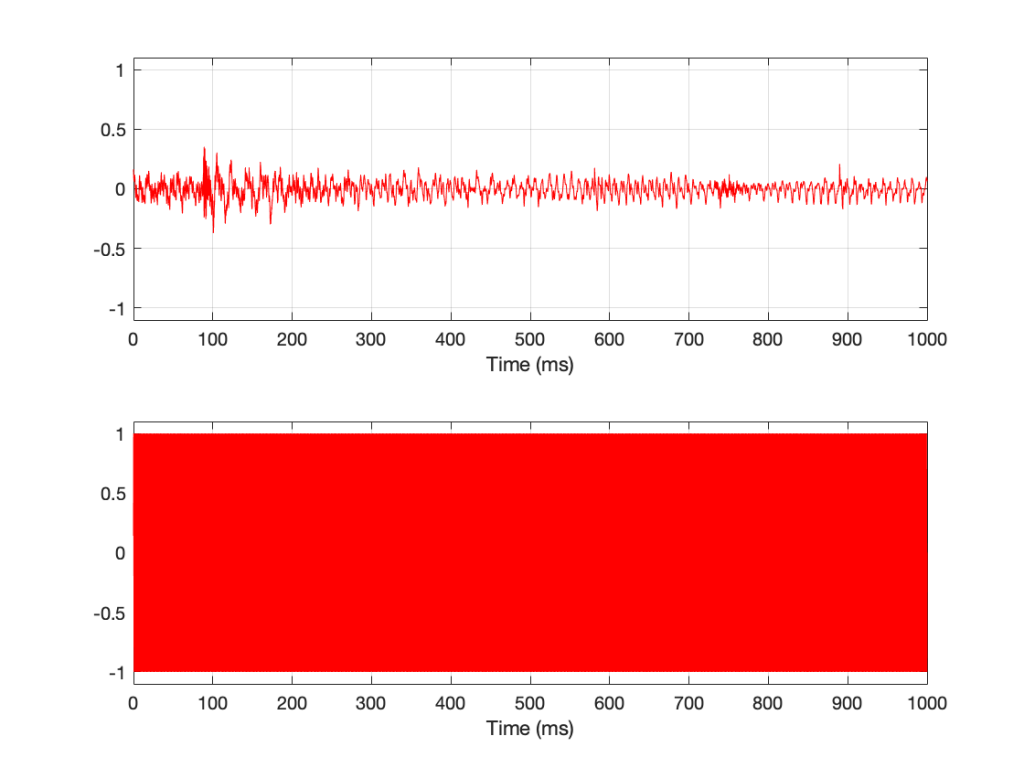Figure 1: The top plot is a 1-second slice from Jennifer Warnes’s “Bird on a Wire”. The bottom plot is a 1-second slice of a 997 Hz sine tone.

Looking at Figure 1, it seems obvious that the level of “Bird on a Wire” changes over time, but the level of a sine wave doesn’t. However, that’s not as obvious when we zoom into that plot, as is shown in Figure 2, below.

From Figure 2, we can easily establish the obvious fact that “Bird on a Wire” and a sine wave are different. However, now it’s not as obvious that the sine wave as a constant level – it repeats itself periodically – which is why we call it “periodic” – but what is its level?

The simplest way to determine the level of a signal is similar to the way yesterday’s share prices are shown in the financial section of the newspaper. In that case, you are told the highest price and the lowest price for the day. In audio, we sometimes talk to the “peak-to-peak” amplitude of a signal. This is the difference between the highest and and the lowest peak (more accurately called a “trough”) of the signal in whatever amount of time you’ve been measuring. For example, take a look at Figure 3.

In Figure 3, I’ve drawn two signals. The top one is a 100 Hz sine wave with a peak-to-peak amplitude of 2 (because the difference between the highest peak (+1) and the lowest peak (-1) is 2). The bottom signal is a 100 Hz sine wave with a peak-to-peak amplitude of 0.1 – but with two clicks – one hitting +1 and the other hitting -1. So, if I just look at the peaks of that second signal, it also has a peak-to-peak amplitude of 2.

So, although it was easy to find the peak-to-peak amplitudes of those two signals, it should be obvious that this does not give a fair indication of how loud they appear to be.

However, if you’re building a piece of audio equipment (like an amplifier or an EQ, for example), this measurement does give you an idea of the “worst case” limits of the signal that might come through the system. So it’s not a useless measurement.

An additional problem with a peak-to-peak measurement of a signal is that it doesn’t tell you anything about asymmetry across the 0 line. (In an analogue world, we’d call that a “DC offset” because there would be a DC voltage that is added to the AC waveform.) For example, both of the signals in Figure 4 have a peak-to-peak amplitude of 1, but they are different…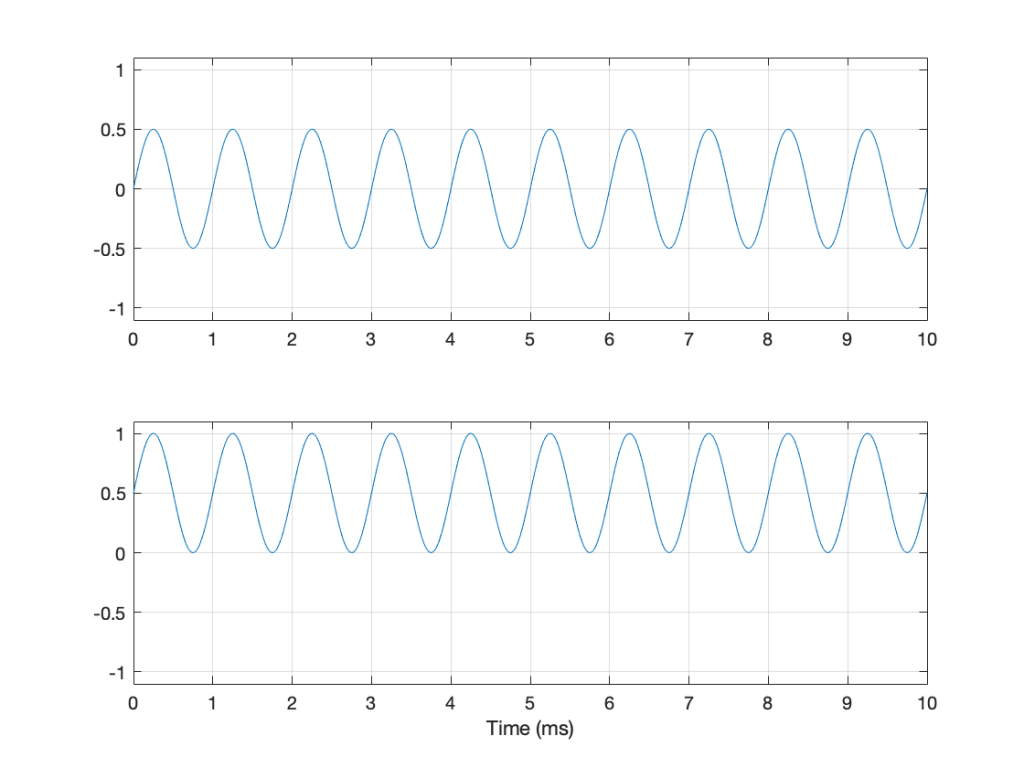Figure 4: Two sine waves with the same frequency and the same peak-to-peak amplitude. One of them will be nice to a loudspeaker driver (the top one…) but the other will not (the bottom one)

If you’re lazy, you can do half of a peak-to-peak measurement. This is where you just check the maximum value of either the peak or the trough. We call this a “peak” amplitude measurement.

This has its problems, though. For example, take a look at Figure 5.

Here, we see two signals. The top one is a sine wave. The bottom one was a sine wave until I squished its negative-going half with a cheap compressor. As you can probably see, the top waveform is symmetrical – the negative half of the signal is the same as the positive half of the signal, just upside-down. It is also easily obvious that the second signal on the lower plot is not symmetrical. Its positive peak is higher than its negative peak.

However, both of these signals have a maximum positive peak of 1 – therefore their peak amplitudes are both 1 (but their peak-to-peak amplitudes and their apparent loudnesses are different).

You might think that an easy way around this problem is to look at the absolute value of the signals and find the peaks that way. However, as you can see in Figure 6, in the case of asymmetrical signals, this does not change anything.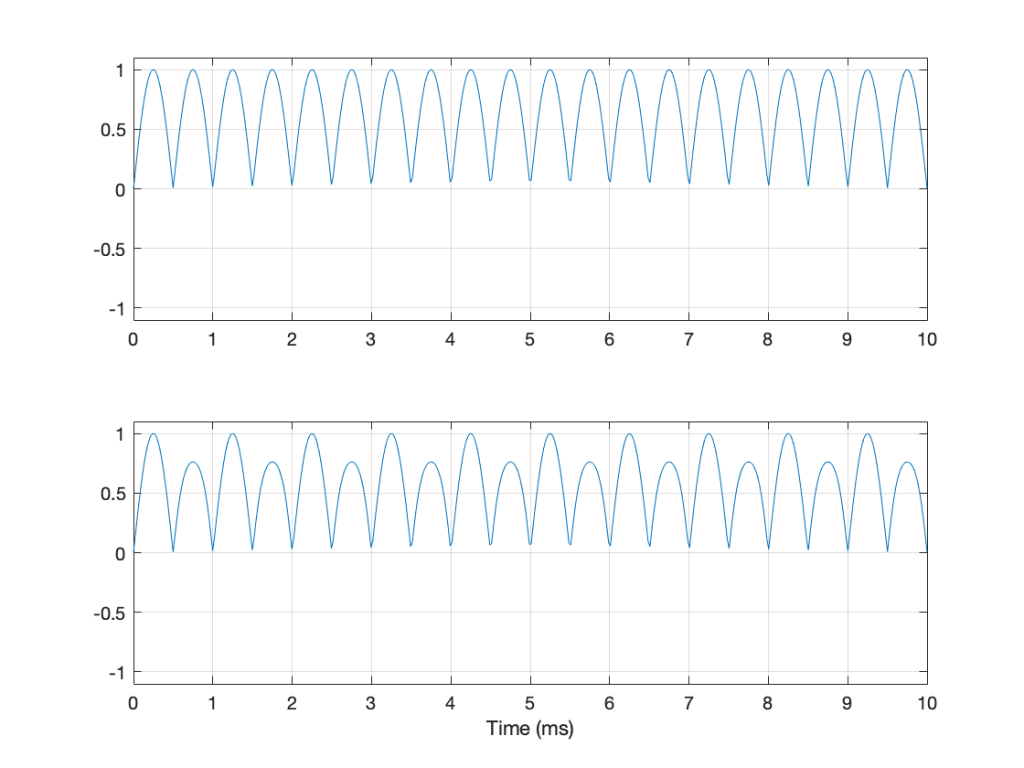Figure 6: The same signals shown in Figure 5 – but the plot shows the absolute values of the signals. Note that their peak amplitudes are still the same here.

Another way to look at the signal is to take an average of the level over time. However, if the signal is symmetrical (like a sine wave, for example) this would not work, since the average will probably be 0. This is because, if the signal is symmetrical, then the average of all of the negative values in the signal (over time) average out to be the negative equal of the average of all of the positive values. So we can’t just use the average of the signal directly… However, with a little extra math, we can do something useful.

I’m going to skip quickly over some old-fashioned math here in order to jump to the punchline which is: “the power in an AC signal (like a sine wave) is proportional to the square of the signal.”

The reason for this can be explained by combining Ohm’s Law and Watt’s Law as follows:

V = IR

where V is electromotive force (or voltage) in volts, I is current in amperes, and R is the resistance in ohms.

P = VI

where P is the power in watts, and V and I are the same as above.

If we fiddle with Ohm’s Law like this:

V = IR

therefore

I = V/R

Then we can replace the “I” in Watt’s Law like this

P = VI

P = V * V/R

P = V2 / R

So, with that last equation, we can see that the Power (in watts) is proportional to the square of the Voltage (in volts). So, if you double the voltage, you get 4 times the power (because 22 = 4).

We could do the same thing for current, as follows:

P = VI

P = IR * I

P = I2 R

So what? Well, one thing this tells us is that, if you want double the power (for example, from a loudspeaker’s output or the heat from a hair dryer) then you’ll need 4 times the amplitude of the signal feeding it (for example, 4 times the voltage at the same current level or 4 times the current with the same voltage).

Now, let’s come back to the problem at hand… What’s the level of the signal? Well, we start by taking our signal and find its equivalent power (by squaring its instantaneous amplitude value over time – so, for example, if it’s a digital signal, we take the value of each sample and multiply it by itself). Part of the effect of this squaring of the signal is that it removes the negative portion of the signal (because a negative number multiplied by a negative number is a positive number).

We then take a slice of time, and average all of the values that we just created by squaring the original values. Now we have the average (or “mean”) power in the signal.

However, we’re not interested in the power of the signal, we’re interested in its “average” amplitude (say, its voltage). So, to get back from power, we take the square root of the average that we just calculated.

By doing all of this, we are finding the Root of the Mean of the Square of the voltage – the RMS level.

If we apply this math to a sine wave, the result will be something like what’s shown in Figure 6.

In Figure 6, the black curve is the original sine wave with a frequency of 100 Hz and a peak amplitude of 1.0 (and no DC offset). The red curve shows the result of squaring all the values in the sine wave (which is why it’s called a “sine squared” wave or sin2 wave). If we find the average of all of the values in the red curve, the result would be 0.5. The square root of 0.5 is approximately 0.707 – which is shown as the blue line in the plot.

So, the RMS value of a sine wave with a peak value of 1 is 0.707. What does this mean? The easiest way to think of this is that if you had an old-fashioned incandescent light bulb and you powered it with a 1Vp (1 Volt Peak) AC voltage sine wave, it would be exactly the same brightness as if you connected it to a 0.707 V DC battery instead. If you wanted to use a battery to power your toaster, and you wanted it to make toast just as quickly as it normally does, then the battery will have to have a voltage that is 0.707 * the peak value of the AC voltage that normally feeds it. (Note that, if you live in North America, then the electrical signal feeding your toaster is 110 V RMS – so you’ll need a 110 V battery. If you live in Europe, then your toaster is fed with 220 V RMS – so you’ll need a 220 V battery. If you live somewhere else, you might need something else… Note that the electrical company has already done the RMS calculation for you…)

So, an RMS measurement of an AC signal tells us what DC value would result in the same power consumption.

There is just one problem: part of the RMS calculation is the “M” part – we are finding the mean of the values over some period of time. The length of time that we’re going to use is easy to choose if it’s a sine wave – we just make sure that the length of time (we call it a “time constant”) is at least as long as one period of the sine wave itself. If it’s smaller, then the RMS value will bob up and down as the sine wave goes up and down.

However, if we’re going to try to use the RMS method to find the level of a music signal, we’re going to have to make some tough choices… For example, let’s find the RMS value of the “Bird on a Wire” sample, using different time constants, shown below.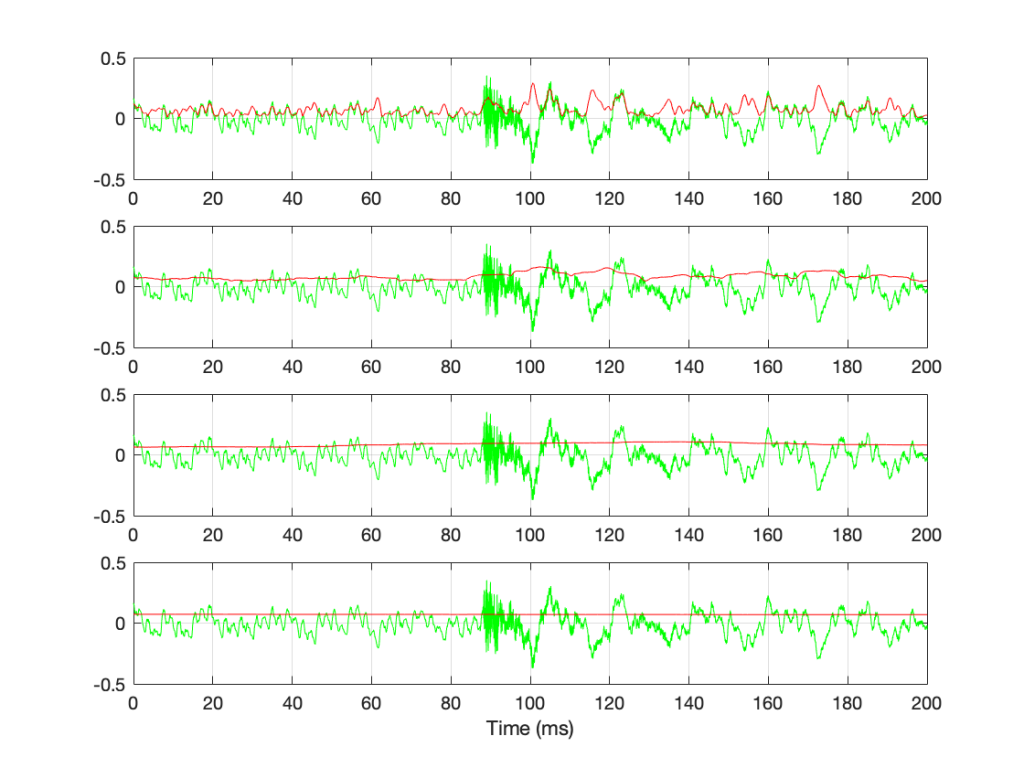Figure 7: The green plots show the same thing: a 200-ms slice out of Bird on a Wire. The red plots show the RMS level of the green signal for 4 different time constants – from top to bottom: 1 ms, 10 ms, 100 ms, and 1 second.

If we convert the plot in Figure 7 to a decibel representation by taking 20*log10 of each sample value, we get the plots in Figure 8. (Note that this is not the same as dB FS, since we are not comparing the result to the RMS value of a full-scale sine wave… but that’s a topic for another posting.)

There are some things that are evident in Figure 8. The most obvious one is that there is a link between the RMS time constant and the variability of the RMS level when the signal that you’re analysing is not periodic. Looking at this short 200 ms-long example from Bird on a Wire, with the four time constants that I used, the range of results are as shown below in Figure 9.

RMS Time constant Min (dB) Max (dB) Range (dB)
Original signal -∞ -9.1
1 ms -43.6 -10.8 32.8
10 ms -27.2 -15.8 11.4
100 ms -24.0 -19.4 4.6
1 sec -23.2 -22.8 0.4

Of course, it’s important to remember that if I had picked a different signal or different RMS time constants, I would have gotten different results.

The question to ask here is:

“If I want to know the level of that 200 ms slice of Bird on a Wire, which RMS time constant should I use?”

or

“which of those four plots tells me the signal’s level?”

The answer is that none of these is correct – or all of them are, even though they show different things. The problem is that music has such a wide frequency range – from 20 Hz to 20,000 Hz. Therefore, if you choose a time constant that is long enough to give you a stable measurement at 20 Hz (which will be at least 50 ms – 1/20th of a second or one period of a 20 Hz wave), then it will be the length of 1000 periods of the 20 kHz portion of the signal.

Of course, you could argue that you care more about the 20 Hz part than the 20,000 Hz part – but that’s dependent on what you’re doing. If you’re measuring the signal that’s being sent to a tweeter, then you’re probably not interested in what’s going on at 20 Hz at all…

## So what?

We’re heading (in a future posting) towards talking about measuring a system’s (or a devices’s) ability to deliver a wide range of signal levels. We’re going to talk about its “signal to noise ratio” which is a measurement of how much louder the signal (the music) is than the noise that the system itself generates. The idea in the design of all audio systems is that you want to make that ratio as big as possible so that you cannot hear the noise because it’s so much quieter than the signal.

The problem is that we’re going to have to measure how loud the signal can be – and compare that to how loud the signal actually is at any given moment. In order to understand the concepts in that discussion, then it’s necessary to understand the concepts that I introduced above, namely the following:

• Peak-peak level
• Peak level
• RMS level
• the relationship between RMS Time constant and the RMS level

# Data visualisation

This past week, I spent two days learning the basics of representing data in Tableau, which led to a discussion about Stephen Few, who has some opinions about clearly representing your data.

That, in turn, got me thinking about one of my old hobby horses – my personal mission to eliminate the world of spider plots.

# Resolution, Part 1: White Noise

This is the start of what will be a series of posts that are an attempt to answer a question about the pro’s and con’s of implementing a volume control in the digital domain. When I first thought about how to answer this question, I thought I could do it in a couple of sentences – but the more I thought about it, the more I realised that the answer is complicated…

There’s no doubt in my mind that I’m making this answer more complicated than necessary, but, as Carl Sagan once said, “If you wish to make apple pie from scratch, you must first create the universe.”

So, to begin, we have to define what “noise” is from the point of view of audio engineering.

On the one hand, we can define it simply. “Noise” is a random signal. We can be more accurate and say that this means that the amplitude of a noise signal cannot be predicted using a knowledge of what has come before in time.

If I flip a coin, it will be either heads or tails. I can’t predict this. It will be random. If I flip it 100 times, and, by some strange coincidence, I get 100 “tails”, there is still a 50% chance of getting a tails on the 101st flip. What has happened before can, in no way, be used to predict what is about to happen.

Of course, what is about to happen on the 101st flip has a limited number of possible outcomes. I cannot flip the coin and get “dog” as a result… (this sounds silly, but it will come in handy later…) Just like I cannot roll two dice and get a 13…

In LPCM digital audio, a noise signal is one where each individual sample in the signal has a random value that is in no way related to any of the previous samples. Its range (the set of possible values from which we can pick our random number) may be limited (depending on the specific characteristics of the noise signal and what may have come before), but it will be random.

Typically, when you are talking to someone in audio about noise, they describe it using a colour as the first descriptor. So, you’ll hear of “white noise” and “pink noise”, as the two most popular examples. For the purposes of this series of postings, we’ll only be talking about white noise. So, what is this?

One definition that you’ll see thrown around a lot says something like “white noise is a random signal that has equal energy per linear bandwidth” or “… equal energy per hertz” or “…equal intensity at different frequencies” or something like this. These descriptions are sort of true if you don’t want to get into temporal details, which, unfortunately, is exactly where we’re headed…

The good thing about those definitions is that they describe a general characteristic of white noise. If you take a white noise signal, and you measure the intensity of (or the energy in) the signal for a given bandwidth (say, a bandwidth of 100 Hz ranging from 200 Hz to 300 Hz) then it will be the same in another frequency range with the same bandwidth (say, a bandwidth of 100 Hz ranging from 1,000 Hz to 1,100 Hz). Note that these two bandwidths are the same in hertz – not in a multiplier like octaves or semitones or decades. So, if you have white noise that has a total bandwidth of 0 Hz to 20,000 Hz, then you will have the same amount of energy in the 0 – to – 10,000 Hz band as you will in the 10,000 – to – 20,000 Hz band. In other words (to us humans), there is as much energy in the top octave of the signal as in the rest of the bandwidth combined.

This is why white noise sounds like “bright” and “hissy” (similar to the “ss” sound in “hissy”) and not “darker” like the “sh” sound in “ash” (as they incorrectly claim here…). Since white is a “bright” colour, then we use the word “white” to describe the frequency-dependent energy distribution of “white” noise.

However, this is not really true. The truth is that a white noise signal has an equal probability per bandwidth of having the same energy level. This little detail is usually left out, partly because it’s complicated, and partly because it doesn’t matter in most cases in the real world. However, in our case, it does.

Let’s look at an example. I made a white noise signal in Matlab using the statement
rand(SignalLength, 1) – rand(SignalLength, 1)
where SignalLength is the length of the noise signal in samples, and the 1 means that I’m doing this for 1 audio channel…. mono is so retro…

You may be wondering why I did a
rand() – rand()
rand().
the simple answer for now was that I wanted to make the signal “balanced” on either side of the zero line and the rand() function in Matlab has a range of 0 to 1.

I know… I could have done this by saying
2 * (rand(SignalLength, 1) – 0.5)
but there is another reason that we’ll get into later…)

I then used a DFT to find the magnitude response of this signal. The result – both the signal and its magnitude response – are shown below in Figure 1.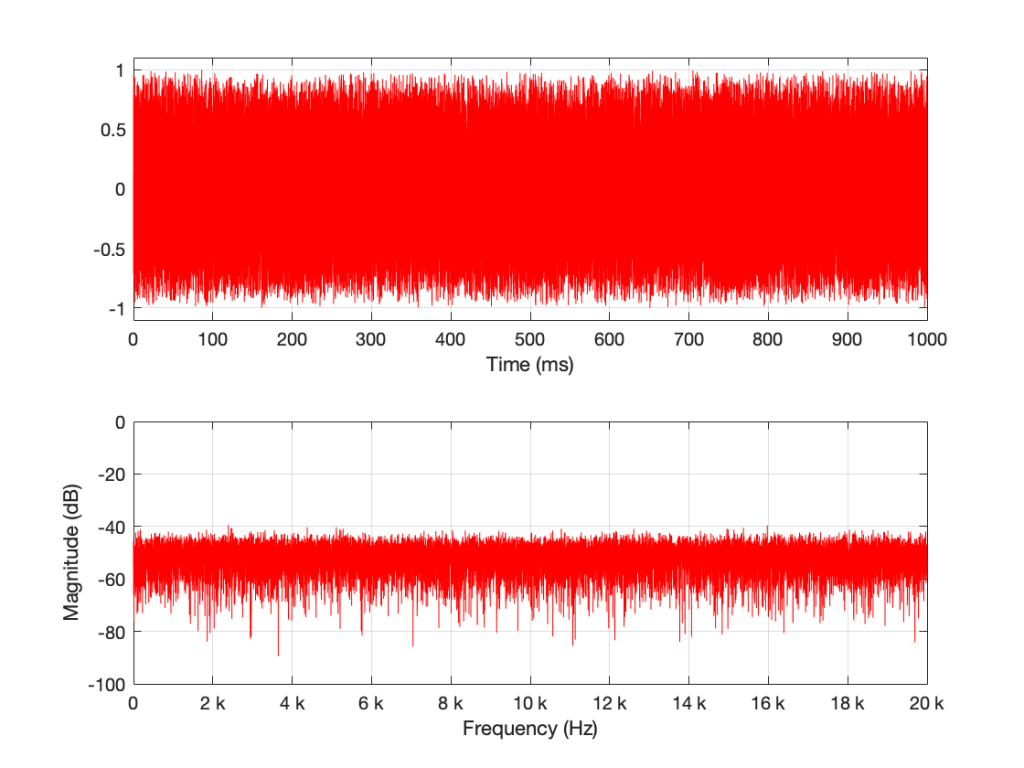Figure 1: A random signal shown in the time domain (top plot) and its magnitude response (bottom plot).

Some additional information that is really not important: The sampling rate of this signal is 2^16 (65,536 Hz), and I did a 2^16 point DFT, so I have one frequency bin per hertz. (If this last bit of information is confusing, but interesting, please start reading this…)

You may notice that the magnitude is “flat” – meaning that it generally doesn’t slope upwards or downwards. However, you will also notice that it is certainly not “flat” – meaning that it is not a perfectly straight line. In fact, if we zoom in on both plots, we can see Figure 2.

Notice that we do NOT have an equal amount of energy per hertz… if we did, then the bottom plot would be a flat line.

If I do all of that again – make a new noise sample the same way (with a new set of random numbers) and plot the result, and a zoomed in version, I get Figures 3 and 4.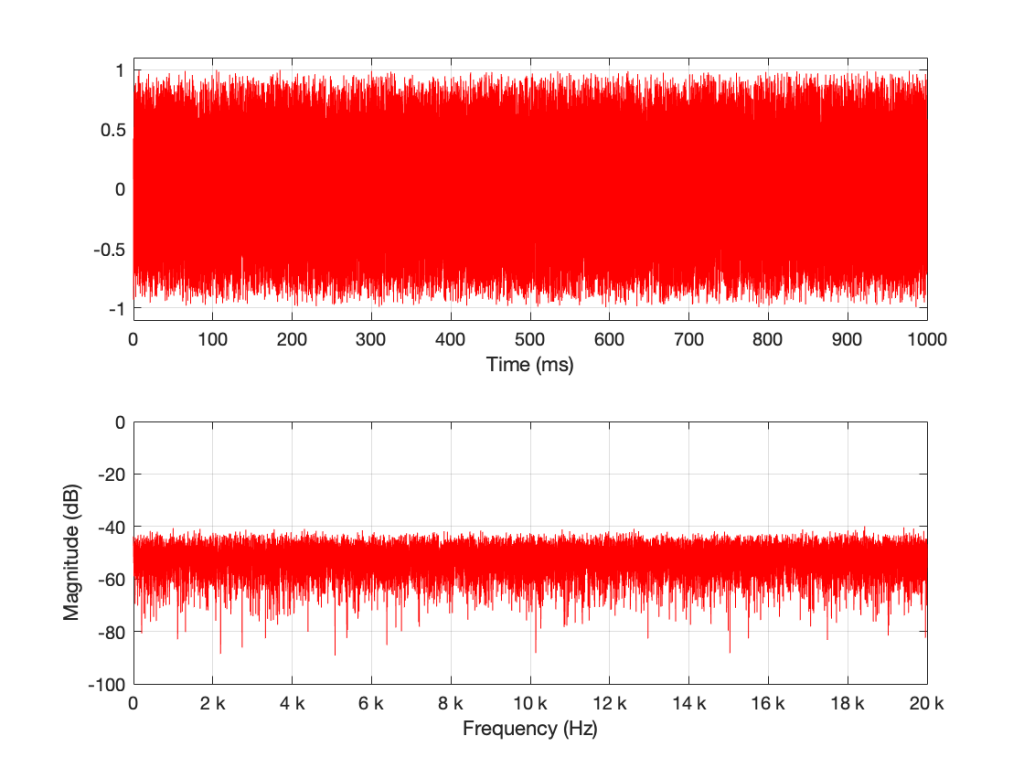Figure 3: The result of running the same code that generated Figure 1. However, this is a new set of random numbers. Notice that, on first glance, it is the same as Figure 1, but if you look carefully, it is completely different.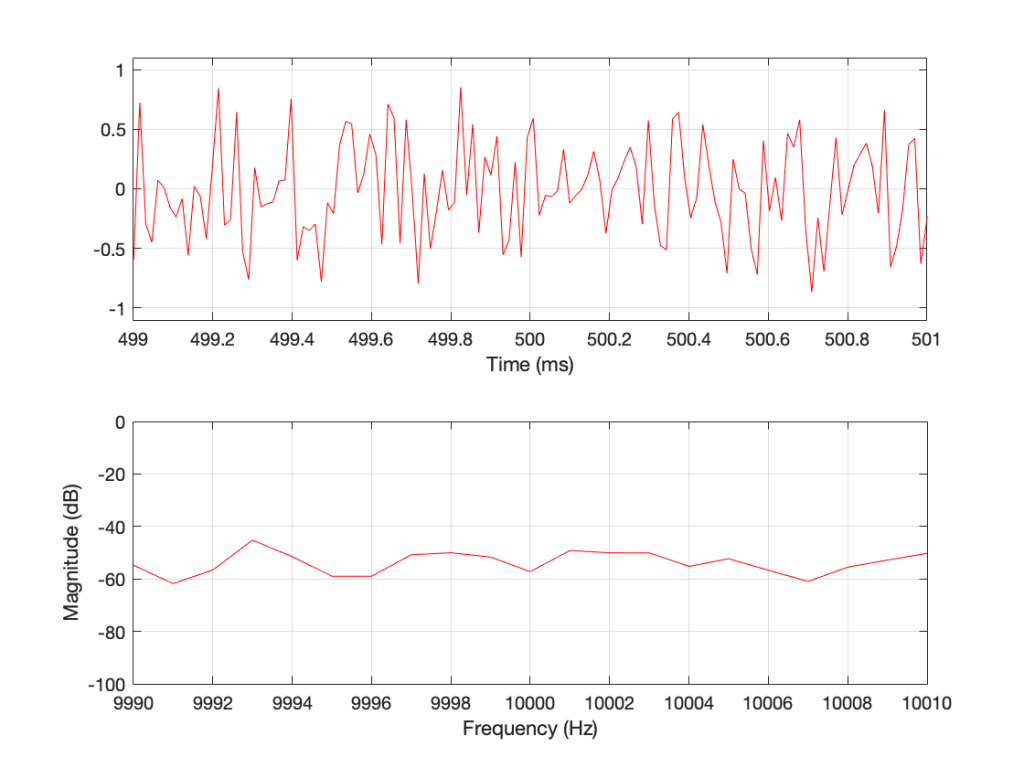Figure 4: A detail from Figure 3. Notice that, on first glance, it is the same as Figure 2, but if you look carefully, it is completely different.

Compare Figures 1 and 3 or Figure 2 and 4. You’ll notice that they have similar characteristics overall – but not only are they NOT identical, they are completely different (on a sample-by-sample or a DFT bin-by-bin comparison).

Let’s say that I run this code and generate a white noise signal 1 second long, and I then calculate the magnitude response of that noise signal and store it. Then, I’ll repeat this, and average the new magnitude response to the first one. Then, I’ll do it again, each time, including the magnitude response to the average of all of the magnitude responses that I’ve done….

For each 1-second slice of time, the noise signal does not have equal energy per bandwidth – however, it is certainly white noise.

This is because, each time I do this, the average magnitude response will get flatter and flatter… and eventually, after doing this an infinite number of times, it will be a flat line.

This means that, white noise will have an equal amount of energy per bandwidth only if I wait long enough. The question is “how long is long enough?” The answer to that question depends on what you’re doing with it.

## Another way to look at this…

In the each of the examples above, I made 1 second-long white noise signals and used the entire signal – all 65,536 samples – to calculate the magnitude response.

What happens if I have a one-second long signal, but only a portion of it is a burst of white noise, and the rest is silence? For example, look at Figure 5.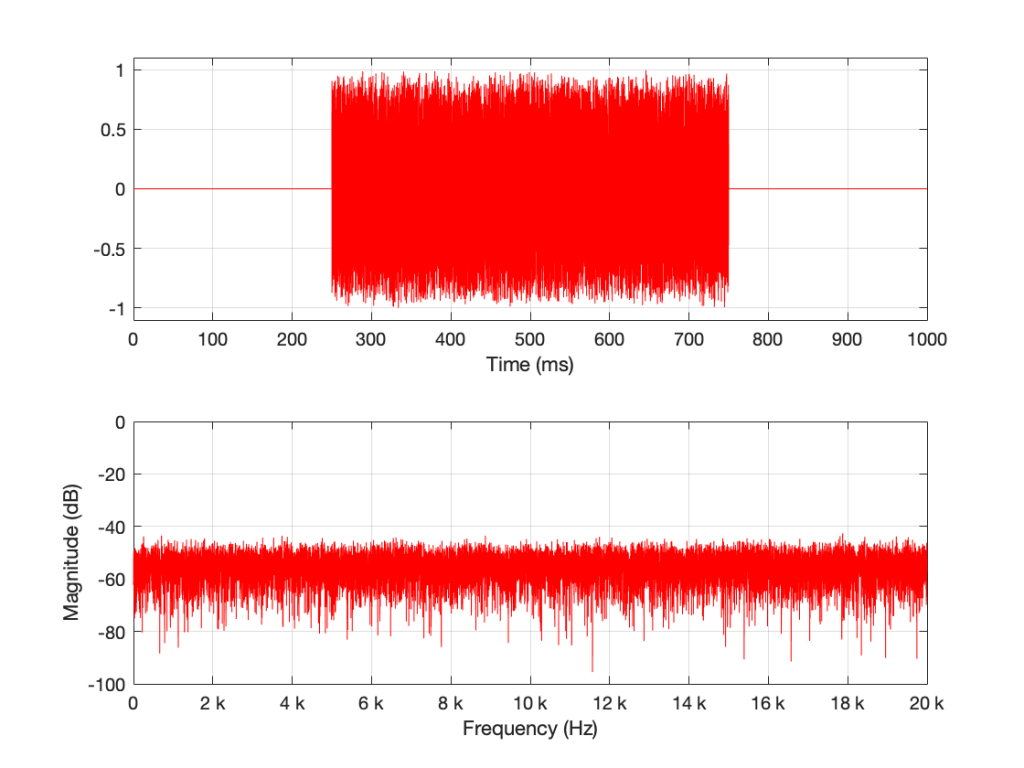Figure 5: A 1-second long signal that contains a burst of white noise for 0.5 seconds.

Figure 5’s magnitude response looks similar to the ones we’ve seen before (apart from being a little lower overall than the plots in Figures 1 and 3 – because there’s less energy overall in 0.5 sec of noise than there is in 1 second of noise). I’ll keep going to show what happens if we take this to an extreme.

The magnitude response shown in Figure 7 looks very different from the ones we’ve seen before. It’s much smoother… We’ll keep going…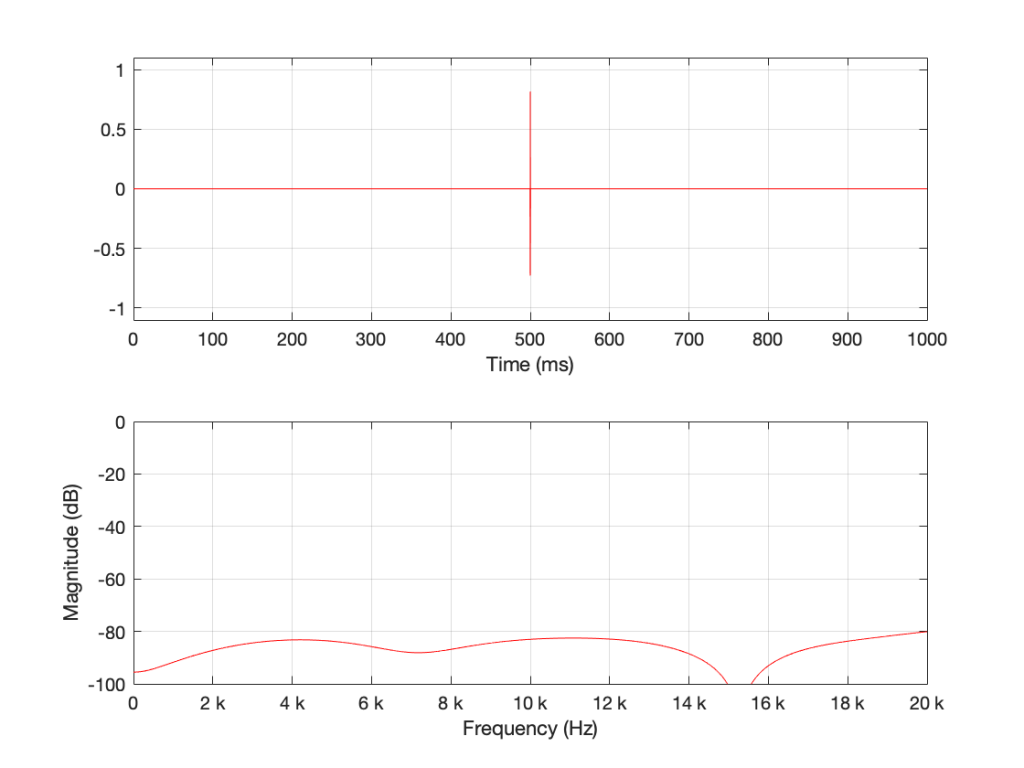Figure 8. 16 samples of white noise in the middle of a 65,536 sample long signal of silence. Notice that, even when not “zoomed in”, the magnitude response is smooth

Figure 8 is very different again… The total magnitude response, even when not “zoomed in” is smooth. It’s important here to note that the actual response that we see there will be different every time I run the random generator again. For example, look at Figure 9, which is also a 16-sample long white noise signal.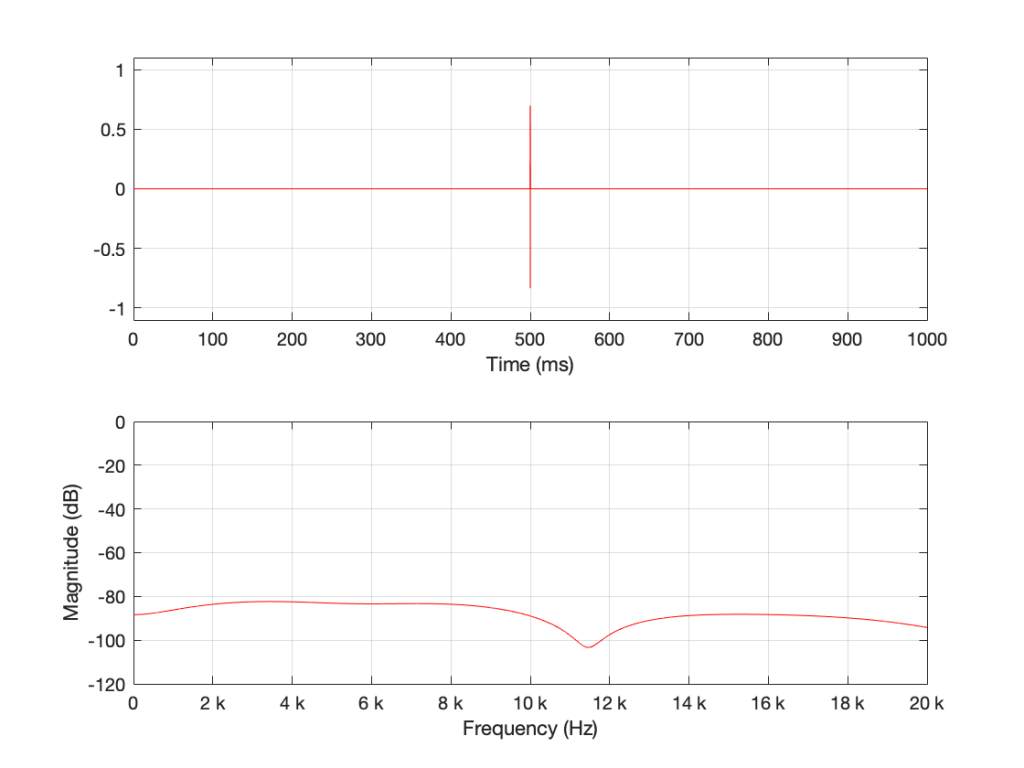Figure 9. 16 samples of white noise in the middle of a 65,536 sample long signal of silence. Notice that, even when not “zoomed in”, the magnitude response is smooth – but it is not identical to the one in Figure 8 because the random numbers are not the same.

If we keep getting shorter and shorter, eventually we’ll get down to a single sample with a random value. However, since it’s a single sample (that is very probably non-zero) in a long string of zeros, then its magnitude response will be completely flat. It will not be noise – it will be an impulse with a random level. And it won’t sound like noise – it will sound like a click.

Summary

There are two basic important things to know at this point.

• White noise has the frequency content you expect only if you average over time.
• The shorter the time the noise is present, the less energy you will have, overall.

The discussion continues in Part 2.

## P.S.

Thanks to David for emailing and pointing out that it’s “Hz” and “hertz” but not “Hertz”. I’ve corrected the text above… Being reminded of this reminds me of a Steven Wright joke – “I’m having amnesia and déjà vu at the same time. I think I’ve forgotten this before…”

# DFT’s Part 6: Windowing artefacts

In Part 5, we talked about the idea of using a windowing function to “clean up” a DFT of a signal, and the cost of doing so. We talked about how the magnitude response that is given by the DFT is rarely “the Truth” – and that the amount that it’s not True is dependent on the interaction between the frequency content of the signal, the signal envelope, the windowing function, the size of the FFT, and the sampling rate. The only real solution to this problem is to know what-not-to-believe when you look at a DFT output.

However, we “only” looked at the artefacts on the magnitude response in the previous posting. In this last posting, we’ll dig a little deeper and NOT throw away the phase information. The problem is that, when you’re windowing, you’re not just looking at a screwed up version of the magnitude response, you’re also looking at a screwed up phase response as well.

We saw in Part 1 and Part 2 how the phase of a sinusoidal waveform can be converted to the sum of a real and an imaginary component. (In other words, if you add a cosine and a sine of the same frequency with very specific separate gains applied to them, the result will be a sinusoidal waveform with any amplitude and phase that you want.) For this posting, we’ll be looking at the artefacts of the same windowing functions that we’ve been working on – but keeping the real and imaginary components separate.

Rectangular windowing

We’ll start by looking at a plot from the previous post, which I’ve duplicated below.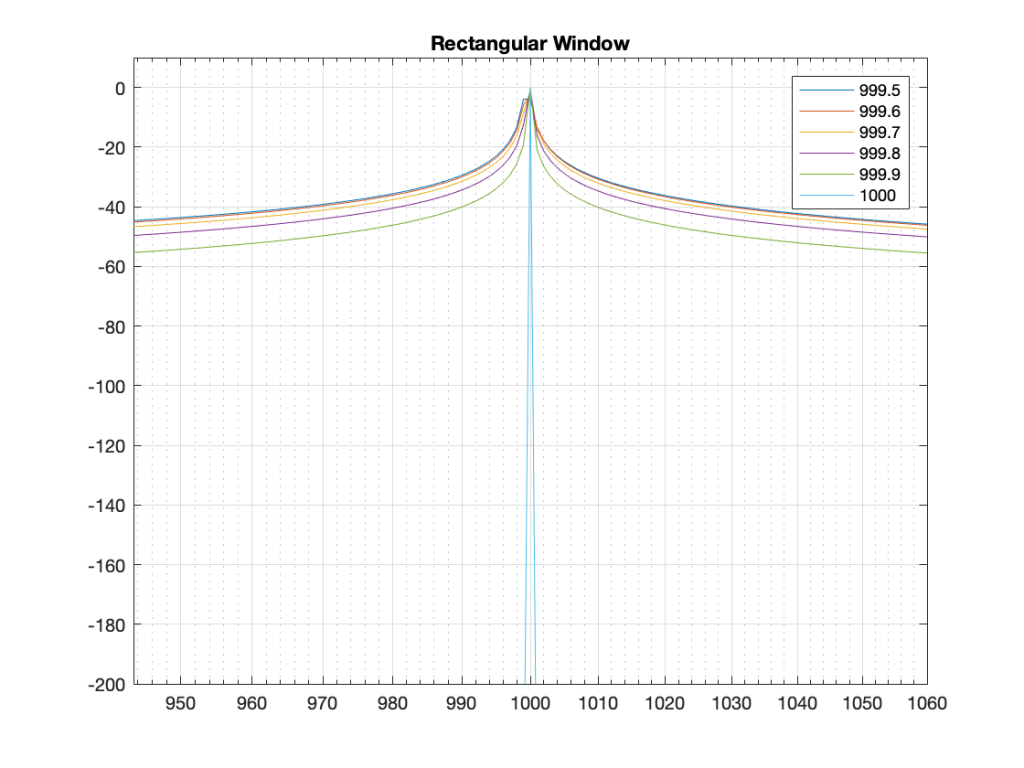Figure 1: The magnitude responses calculated by a DFT for 6 different frequencies. Note that the bin centre frequency is 1000.0 Hz.

The way I did the plot in Figure 1 was to create a sine wave with a given frequency, do a DFT of that, and plot the magnitude of the result. I did that for 6 different frequencies, ranging from 1000 Hz (exactly on a bin centre frequency) to 999.5 Hz (halfway to the adjacent bin centre frequency).

There’s a different way to plot this, which is to show the result of the DFT output, bin by bin, for a sinusoidal waveform with a frequency relative to the bin centre frequency. This is shown below in Figure 2.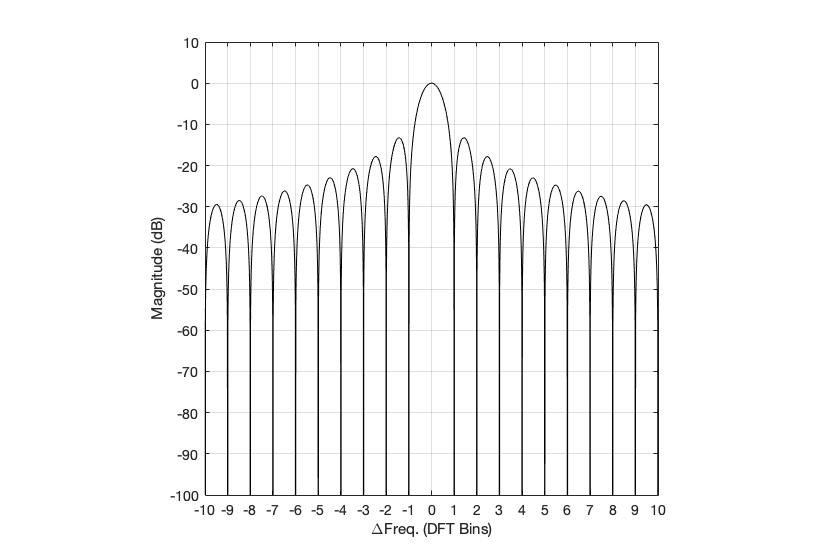Figure 2: Rectangular window: The relationship between the frequency of the signal, the frequency centres of the DFT bin, and the resulting magnitude in dB. Note that the X-axis is frequency, measured in distance between bin frequencies or “bin widths”.

Now we have to talk about how to read that plot… This tells me the following (as examples):

• If the bin centre frequency EXACTLY matches the frequency of the signal (therefore, the ∆ Freq. = 0) then the magnitude of that bin will be 0 dB (in other words, it will give me the correct answer).
• If the bin centre frequency is EXACTLY an integer number of bin widths away from the frequency of the signal (therefore, the ∆ Freq. = … -10, -9, – 8… -3, -2, -1, 1, 2, 3, … 8, 9, 10, …) then the magnitude of that bin will be -∞ dB (in other words, it will have no output).
• These two first points are why the light blue curve is so good in Figure 1.
• If the frequency of the signal is half-way between two bins (therefore, the ∆ Freq. = -0.5 or +0.5), then you get an output of about -4 dB (which is what we also saw in the blue curve in Figure 25 in Part 5.
• If the frequency of the signal is an integer number away from half-way between two bins (for example, ∆ Freq. = -2.5, -1.5, 1.5, or 2.5, etc… ) then the output of that bin will be the value shown at the tops of those bumps in the plots… (For example, if you mark a dot at each place where ∆ Freq. = ±x.5 on that curve above, and you join the dots, you’ll get the same curve as the curve for 999.5 Hz in Figure 1.)

So, Figure 2 shows us that, unless the signal frequency is exactly the same as the bin centre frequency, then the DFT’s magnitude will be too low, and there will be an output from all bins.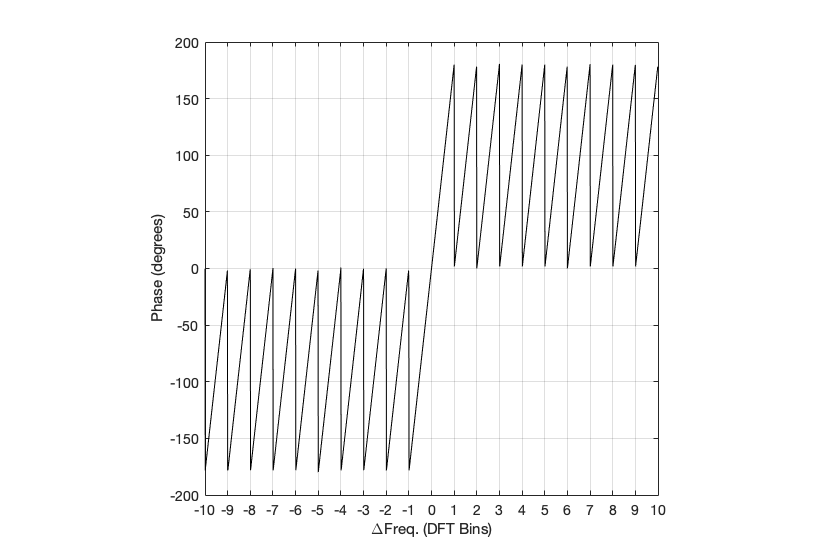Figure 3: Rectangular window: The relationship between the frequency of the signal, the frequency centres of the DFT bin, and the resulting phase in degrees.

Figure 3 shows us the same kind of analysis, but for the phase information instead. The important thing when reading this plot is to keep the magnitude response plot in mind as well. For example:

• when the bin frequency matches the signal frequency (∆ Freq. = 0) then the phase error is 0º.
• When the signal frequency is an integer number of bin widths away from the bin frequency, then it appears that the phase error is either 0º or ±180º, but neither of these is true, since the output is -∞ dB – there is no output (remember the magnitude response plot).
• There is a gradually increasing error from 0º to ±180º (depending on whether you’re going up or down in frequency)( as the signal frequency moves from being adjacent to one bin or the next.
• When you signal frequency crosses the bin frequency, you get a polarity flip (the vertical lines in the sawtooth shape in the plot).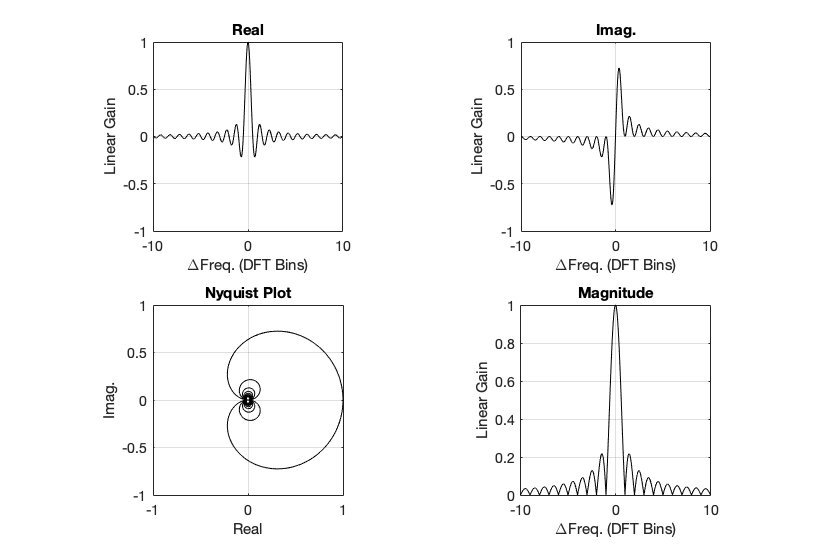Figure 4. Rectangular window: The top two plots show the same relationship as in Figures 2 and 3, but divided into the various components, as explained below.

Figure 4, above, shows the same information, plotted differently.

• The bottom right plot shows the magnitude response (exactly the same as shown in Figure 2) on a linear scale instead of in dB.
• The top two plots show the Real and Imaginary components, which, combined, were used to generate the Magnitude and Phase plots. (Remember from Parts 1 and 2 that the Real component is like looking at the response from above, and the Imaginary component is like looking at the response from the side.)
• The Nyquist plot is difficult, if not impossible to understand if you’ve never seen one before. But looking at the entire length of the animation in Figure 5, below, should help. I won’t bother explaining it more than to say that it (like the Real vs. Freq. and the Imaginary vs. Freq. plots) is just showing two dimensions of a three-dimensional plot – which is why it makes no sense on its own without some prior knowledge.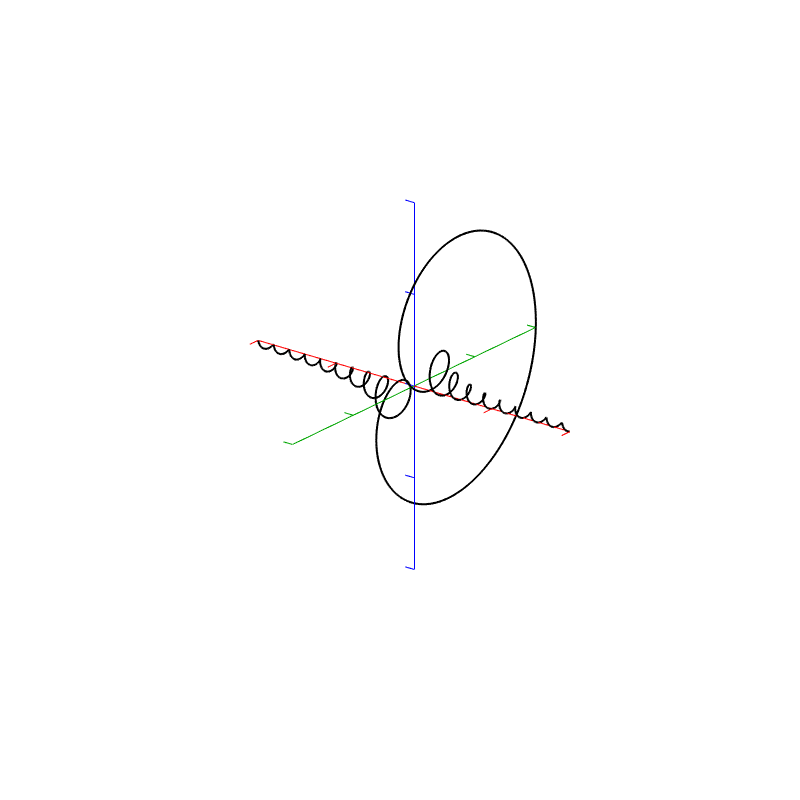Figure 5. Rectangular window:The Real, Imaginary, and Nyquist plots from Figure 4, viewed from different angles.

Hopefully, I’ve said enough about the plots above that you are now equipped to look at the same analyses of the other windowing functions and draw your own conclusions. I’ll just make the occasional comment here and there to highlight something…

## Hann Window

Generally, the things to note with the Hann window are the wider centre lobe, but the lower side lobes (as compared to the rectangular windowing function).

## Hamming window

The interesting thing about the Hamming window is that the lobes adjacent to the main lobe in the middle are lower. This might be useful if you’re trying to ignore some frequency content next to your signal’s frequency.

## Blackman Window

The Blackman window has a wider centre lobe, but the side lobes are lower in level.

## Blackman Harris window

Although the Blackman-Harris window results in a wider centre lobe, as you can see in Figure 18, the side lobes are all at least 90 dB down from that…

## Wrapping up

I know that there’s lots left out of this series on DFT’s. There are other windowing functions that I didn’t talk about. I didn’t look at the math that is used to generate the functions… and I just glossed over lots of things. However, my intention here was not to do a complete analysis – it was a just an introductory discussion to help instil a lack of trust – or a healthy suspicion about the results of a DFT (or FFT – depending on how fast you do the math….).

Also, a reason I did this series was as a set-up, so when I write about some other topics in the future (like the actual resolution of 16-bit LPCM audio in a fixed point world, or the implications of making a volume control in the digital domain as just two examples…), I can refer back to this, pointing out what you can and cannot believe is the plots that I haven’t even made yet…

# DFT’s Part 5: Windowing

The previous posting in this series showed that, if we just take a slice of audio and run it through the DFT math, we get a distorted view of the truth. We’ll see the frequencies that are in the audio signal, but we’ll also see that there’s energy at frequencies that don’t really exist in the original signal. These are artefacts of slicing a “window” of time out of the original signal.

Let’s say that I were a musician, making samples (in this sentence, the word “sample” means what it means to musicians – a slice of a recording of a sound that I will play using a sampler) to put into my latest track in my new hip hop album. (Okay, I use the word “musician” loosely here… but never mind…) I would take a sample – say, of the bell that I recorded, which looks like this:

We’ve already seen that the first sample (now I’m back to using the technical definition of the word “sample” – an instantaneous measurement of the amplitude of the signal) and the last sample aren’t on the 0 line. So, if we just play this recording, it will start and end with a “click”.

We get rid of the click by applying a “fade” on the start and the end of the recording, resulting in something like the following: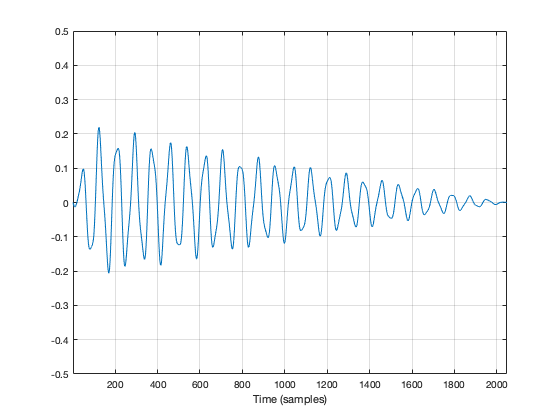Figure 2: The same recording with a fade in and a fade out applied to it. Now it will sound more like a bell because it has a fast attack (a short fade in) and a longer decay (fade out).

So, the moral of the story so far is that, in order to get rid of an audible “click”, we need to fade in and fade out so that we start and end on the 0 line.

We can do the same thing to our slice of audio (from now on, I’m going to call it a “window”) to help the computer that’s doing the DFT math so that it doesn’t get all the extra frequency content caused by the clicks.

In Figure 2, above, I was being artistic with my fade in and fade out. I made the fade in fast (100 samples long) and I made the fade in slow (2000 samples long) so that the end result would look and sound more like a bell. A computer doesn’t care if we’re artistic or not – we’re just trying to get rid of those clicks. So, let’s do it.

Option 1 is to do nothing – which is what we’ve done so far. We take all of the individual samples in our window, and we multiply them by 1. (All other samples (the ones that we’re not using because they’re outside the window) are multiplied by 0.) If you think about what this looks like if I graph it in time, you’ll imagine a rectangle with a height of 1 and a length equal to the length of the window in samples.

If I do that to my original bell recording, I get the result shown in Figure 3.

You may notice that Figure 3 is almost identical to Figure 1. The only difference is that I put the word “Rectangular” at the top. The reason for this will become clear later.

As we’ve already seen, this rectangular windowing of our recording is what gives us the problems in the first place. So, if I do a DFT of that window, I’ll get the following magnitude response.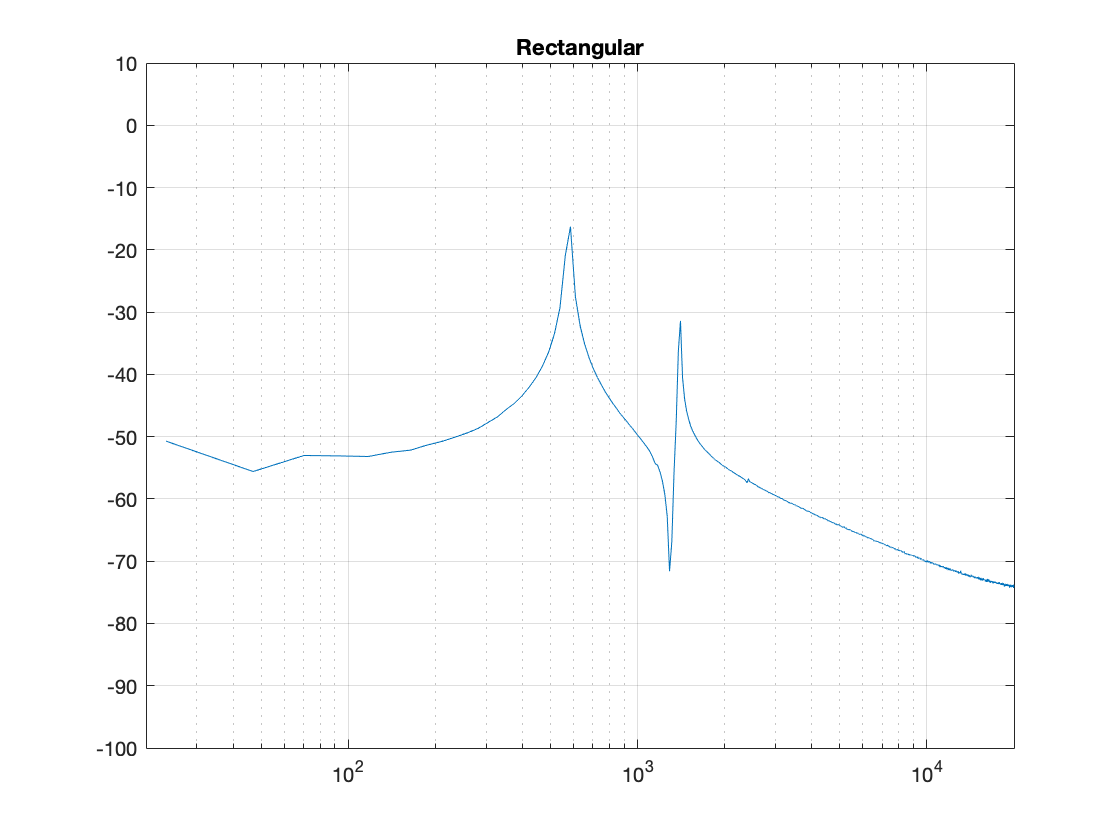Figure 4. The magnitude response of the bell sound with a rectangular window, as shown in Figure 3.

What we know already is that we want to fade in and fade out to get rid of those clicks. So, let’s do that.

Figure 5, above, shows the result. the ramp in and the ramp out are not straight lines – in fact, they look very much like the shape of an upside-down cosine wave (which is exactly what they are…). If we do a DFT of that window, we get the result shown in Figure 6.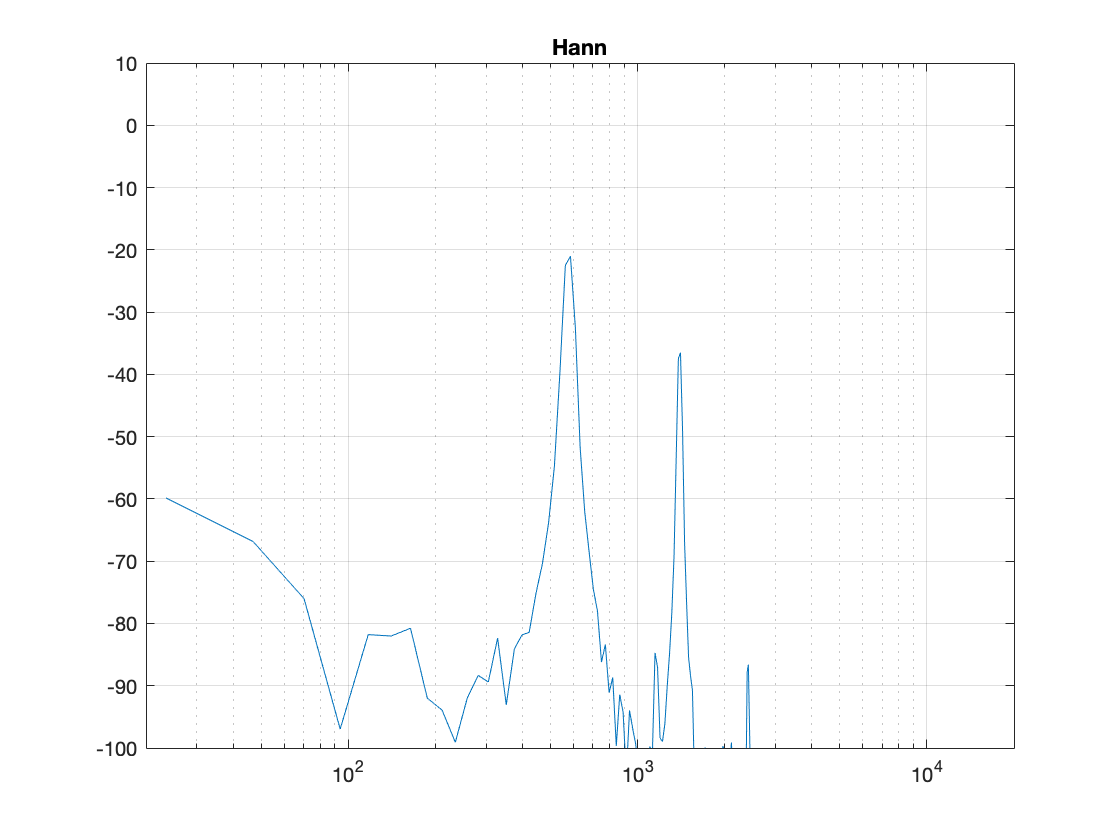Figure 6. The magnitude response of the bell sound with a Hann window, as shown in Figure 5.

There are now some things to talk about.

The first is to say that the shape of this “envelope” or “windowing function” – the gain over time that we apply to the audio signal – is named after the Austrian meteorologist Julius von Hann. He didn’t invent this particular curve – but he did come up with the idea of smoothing data (but in his case, he was smoothing meteorological data over geographical regions – not audio signals over time). Because he came up with the general idea, they named this curve after him.

Important sidebar: Some people will call this a “Hanning” window. This is not strictly correct – it’s a Hann function. However, you can use the excuse that, if you apply a Hann window to the signal, then you are hanning it… which is the kind of obfuscated back-pedalling and revisionist history used to cover up a mistake that is typically only within the purview of government officials.

The second thing is to notice is that the overall response at frequencies that are not in the original signal has dropped significantly. Where, in Figure 4, we see energy around -60 dB FS at all frequencies (give or take….) the plot in Figure 6 drops down below -100 dB FS – off the plot. This is good. It’s the result of getting rid of those non-zero values at the start and stop of the window… No clicks means no energy spread all over the frequency spectrum.

The third thing to discuss is the levels of the peaks in the plots. Take a look at the highest peak in Figure 4. It’s about -17 dB FS or so. The peak at the same frequency in Figure 6 is about -21 dB or so… 4 dB lower… This is because, if you look at the entire time window, there is indeed less energy in there. We made portions of the signal quieter, so, on average, the whole thing is quieter. We’ll look at how much quieter later.

This is where things get a little interesting, because some people think that the way that we faded in and faded out of the window (specifically, using a Hann function) can be improved in some way or another… So, let’s try a different way.

Figure 7, above, shows the same bell sound again, this time processed using a Hamming function instead. It looks very similar to the Hann function – but it’s not identical. For starters, you may notice that the start and stop values are not 0 – although they’re considerably quieter than they would have been if we had used a rectangular windowing function (or, in other words “done nothing”). The result of a DFT on this signal is shown in Figure 8.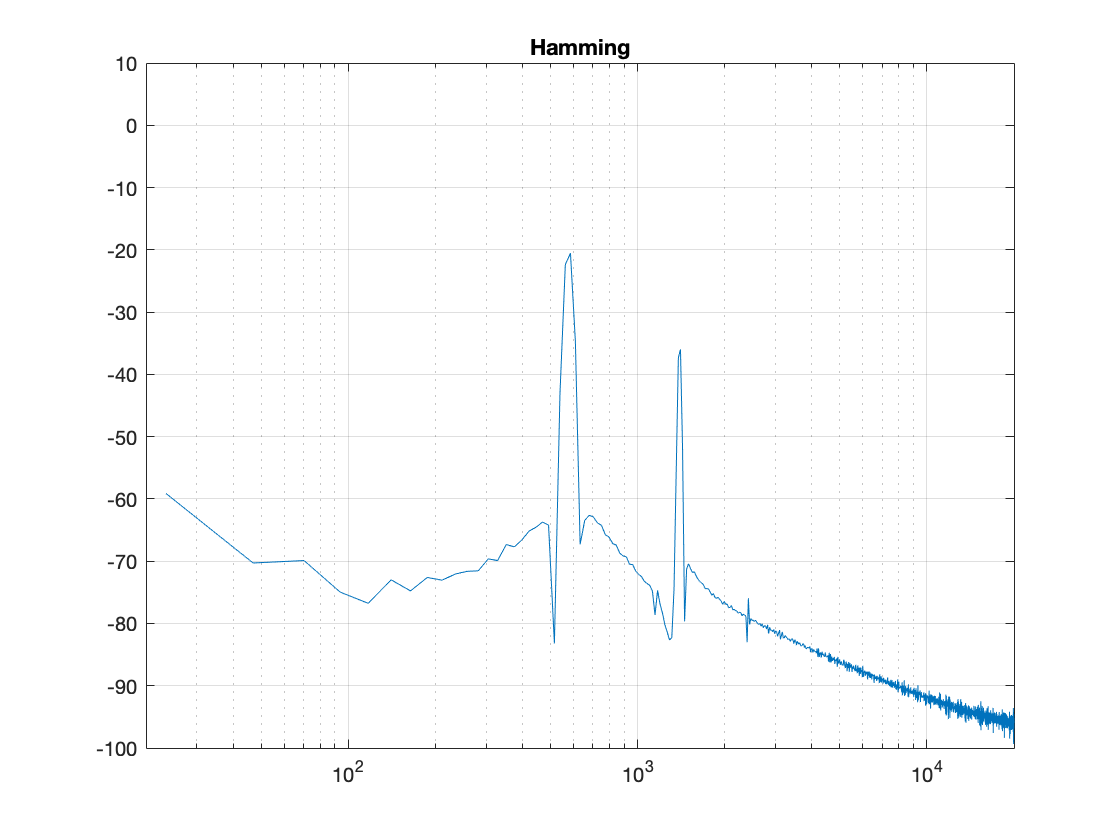Figure 8: The magnitude response of the bell sound with a Hamming window, as shown in Figure 7.

There are two things about Figure 8 that are different from Figure 6. The first is that the overall apparent level of the wide-band artefacts is higher (although not as high as that in Figure 4…). This is because we have a “click” caused by the fact that we don’t start and stop at 0. However, the advantage of this function is that the peaks are narrower – so we get a better idea of the actual signal – we just need to learn to ignore the bottom part of the plot.

Figure 9, shows yet another function, called a Blackman function.

You can see there that it takes longer for the signal to ramp in from 0 (and to ramp out again at the end), so we can expect that the peaks will be even lower than those for the Hann window. This can be seen in Figure 10.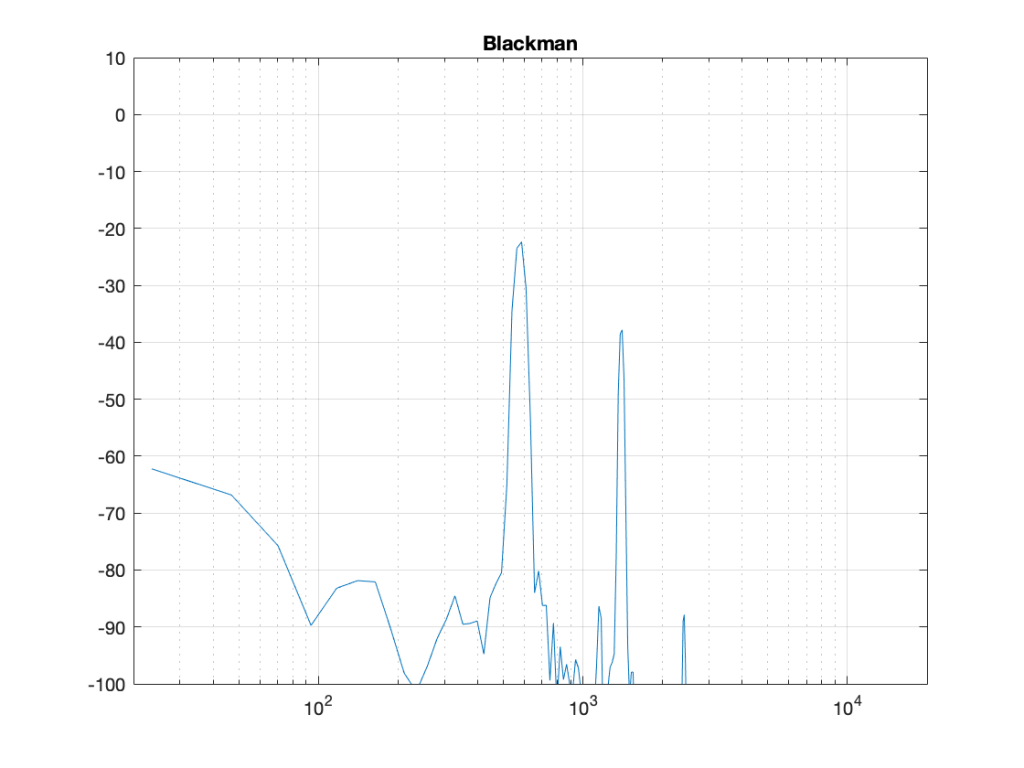Figure 10: The magnitude response of the bell sound with a Blackman window, as shown in Figure 7.

Indeed, the peaks are lower….

Another function is called the Blackman-Harris function, shown in Figures 11 and 12.

There are other windowing functions. And there are some where you can change some variables to play with width and things. Or you can make up your own. I won’t talk about them all here… This is just a brief introduction…

The purpose of this is to show some basic issues with windowing. You can play with the windowing function, but there will be subsequent effects in the DFT result like:

• the apparent magnitude of the actual signal (the peaks in the plots above)
• the apparent magnitude in frequency bands that aren’t in the signal
• the apparent width of the frequency band of the actual signal

Also, you have to remember that a DFT shows you the complete frequency content of the slice of time that you fed it. So, if the frequency content changes over time (the sound of a sitar string being plucked, or the “pew pew” sound of Han Solo’s laser, for example) then this change over time will not be shown…

## Some more details…

Let’s dig a little into the differences in the peaks in the DFT plots above. As we saw in Part 4, if the frequency of the signal you’re analysing is not exactly the same as the frequency of the DFT bin, then the energy will “bleed” into adjacent bins. The example I showed in that posting compared the levels shown by the DFT when the frequency of the signal is either exactly the same as a frequency bin, or half-way between two of them – a reminder of this is shown below in Figure 13.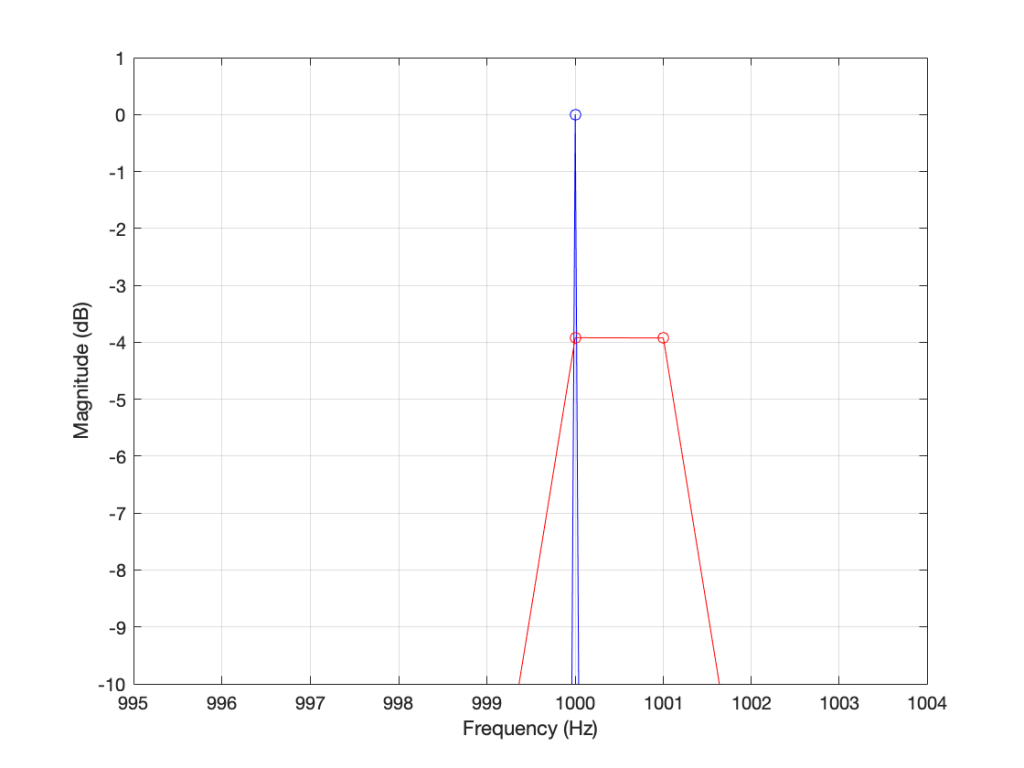Figure 13. The results of a DFT analysis of two signals. The blue plot shows the result when the signal frequency is 1000.0 Hz (exactly the same as the DFT bin frequency). The red plot shows the result when the signal frequency is 1000.5 Hz (half-way between two bins).

As you can see in that plot, the energy in the 1000.5 Hz bleeds into the two adjacent bins. In fact, it’s more accurate to say that there is energy in all of the DFT bins, due to the discontinuity of the signal when the beginning is wrapped around to meet its end.

So, let’s analyse this a little further. I’ll create a signal that is on a frequency bin (therefore it’s a sine wave with a carefully-chosen frequency), and do the DFT. Then, I’ll make the frequency a little lower, and do the DFT again. I’ll repeat this until I get to a signal frequency that is half-way between two bins. I’ll stop there, because once I pass the half-way point, I’ll just start seeing the same behaviour. The result of this is shown for a rectangular window in Figure 14.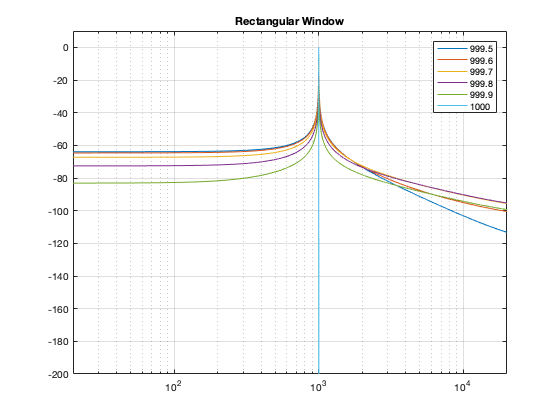Figure 14. The results of doing a DFT analysis on a sine wave with frequencies ranging from exactly on a DFT bin (1000 Hz) to half-way between two bins (999.5 Hz).

As you can see there, there is a LOT of energy bleeding into all frequency bins when the signal is not exactly on a bin. Remember that this does not mean that those frequencies are in the signal – but they are in the signal that the DFT is being asked to analyse.

Let’s do this again for the other windowing functions.

What you may notice when you look at Figures 14 to 18 is that there is a relationship between the narrowness of the plot when the signal is on a bin frequency and the amount of energy that’s spread everywhere else when it’s not. Generally, you have to make a trade between accuracy and precision at the frequency where there’s energy to truth at all other frequencies.

However, if you look carefully at those plots around the 1000 Hz area, you can see that it’s a little more complicated. Let’s zoom into that area and have a look…

The thing to compare in plots 19 to 22 is the how similar the plots in each figure is, relative to each other. For example, in Figure 19, the six plots are very different from each other. In Figure 22, the six plots are almost identical from 995 Hz to 1005 Hz.

Depending on what kind of analysis you’re doing, you have to decide which of these behaviours is most useful to you. In other words, each type of windowing function screws up the result of the DFT. So you have to choose which one screws it up the least for the type of signal and the type of analysis you’re doing.

Alternatively, you can choose a favourite windowing function, and always use that one, and just get used to looking at the way your results are screwed up.

## Some final details

So far, I have not actually defined the details of any of the windowing functions we’ve looked at here. I’ve just said that they fade in and fade out differently. I won’t give you the mathematical equations for creating the actual curves of the functions. You can get that somewhere else. Just look them up on the Internet. However, we can compare the shapes of the gain functions by looking at them on the same plot, which I’ve put in Figure 23.

You may notice that I left out the rectangular window. If I had plotted it, it would just be a straight line of 1’s, which is not a very interesting shape.

What may surprise you is how similar these curves look, especially since they have such different results on the DFT behaviour.

Another way to look at these curves (which is almost never shown) is to see them in decibels instead, which I’ve done in Figure 24.

The reason I’ve plotted them in dB in Figure 24 is to show that, although they all look basically the same in Figure 23, you can see that they’re actually pretty different… For example, notice that, at about 10% of the way into the time of the window, there is a 40 dB difference between the Blackman Harris and the Hann functions… This is a lot.

One thing that I’ve only briefly mentioned is the fact that the windowing functions have an effect on the level that is shown in the DFT result, even when the signal frequency is exactly the same as the DFT bin frequency. As I said earlier, this is because there is, in fact, less energy in the time window overall, because we made the signal quieter at the beginning and end. The question is: “exactly how much quieter?” This is shown in Figure 25.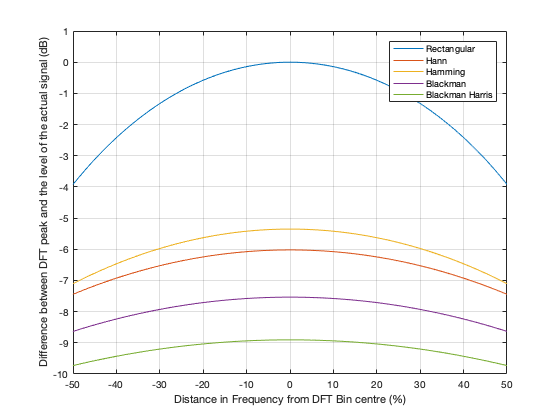Figure 25. The relationship between the signal frequency, the maximum level shown in the DFT results, and the windowing function used.

So, as you can see there, a DFT of a rectangular windowed signal can show the actual level of the signal if the frequency of the signal is exactly the same as the DFT bin centre. All of the other windowing functions will show you a lower level.

HOWEVER, all of the other windowing functions have less variation in that error when the signal frequency moves away from the DFT bin. In other words, (for example) if you use a Blackman Harris window for your DFT, the level that’s displayed will be more wrong than if you used a rectangular window, but it will be more consistent. (Notice that the rectangular window ranges from almost -4 dB to 0 dB, whereas the Blackman Harris window only ranges from about -10 to -9 dB.)

We’ll dig into some more details in the next and final posting in this series… with some exciting animated 3D plots to keep things edu-taining.

# Leon Theremin and RFID

There was an article in the BBC News webpage this week, telling the story of how Leon Theremin (inventor of one of the first electronic musical instruments) invented the technology underneath what we now call RFID…

Of course, this means that every time I swipe my card to buy something at the store, I’m going to start humming the hook from”Good Vibrations”… Maybe knowledge is not always a good thing… Or maybe I should get out my Clara Rockmore album and have another listen.

# DFT’s Part 4: The Artefacts

The previous post ended with the following:

And, you should be left with a question… Why does that plot in Figure 12 look like it’s got lots of energy at a bunch of frequencies – not just two clean spikes? We’ll get into that in the next posting.

Let’s begin by taking a nice, clean example…

If my sampling rate is 65,536 Hz (2^16) and I take one second of audio (therefore 65,536 samples) and I do a DFT, then I’ll get 65,536 values coming out, one for each frequency with an integer value (nothing after the decimal point. The frequencies range from 0 to 65,535 Hz, on integer values (so, 1 Hz, 2 Hz, 3 Hz, etc…) (And we’ll remember to throw away the top half of those values due to mirroring which we talked about in the last post.)

I then make a sine wave with an amplitude of 0 dB FS and a frequency of 1,000 Hz for 1 second, and I do an DFT of it, and then convert the output to show me just the magnitude (the level) of the signal (so I’m ignoring phase). The result would look like the plot below.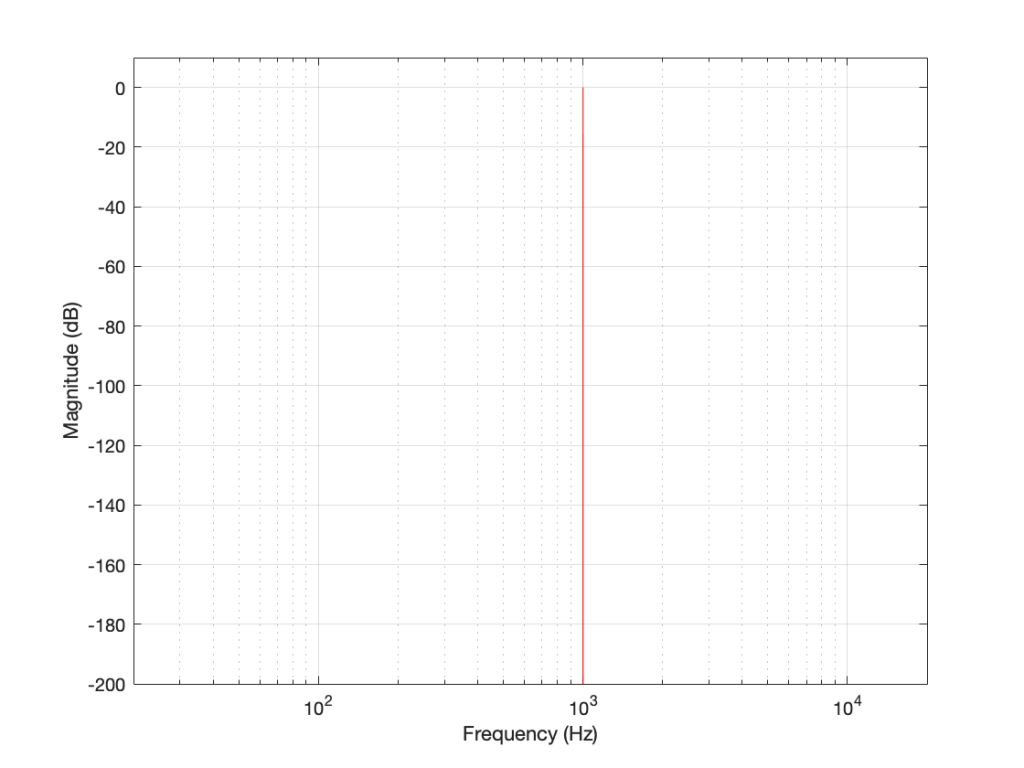Figure 1. The magnitude response of a 1000 Hz sine tone, sampled at 65,536 Hz, calculated using a 65,536-point DFT.

The plot above looks very nice. I put in a 1,000 Hz sine wave at 0 dB FS, and the plot tells me that I have a signal at 1,000 Hz and 0 dB FS and nothing at any other frequency (at least with a dynamic range of 200 dB). However, what happens if my signal is 1000.5 Hz instead? Let’s try that: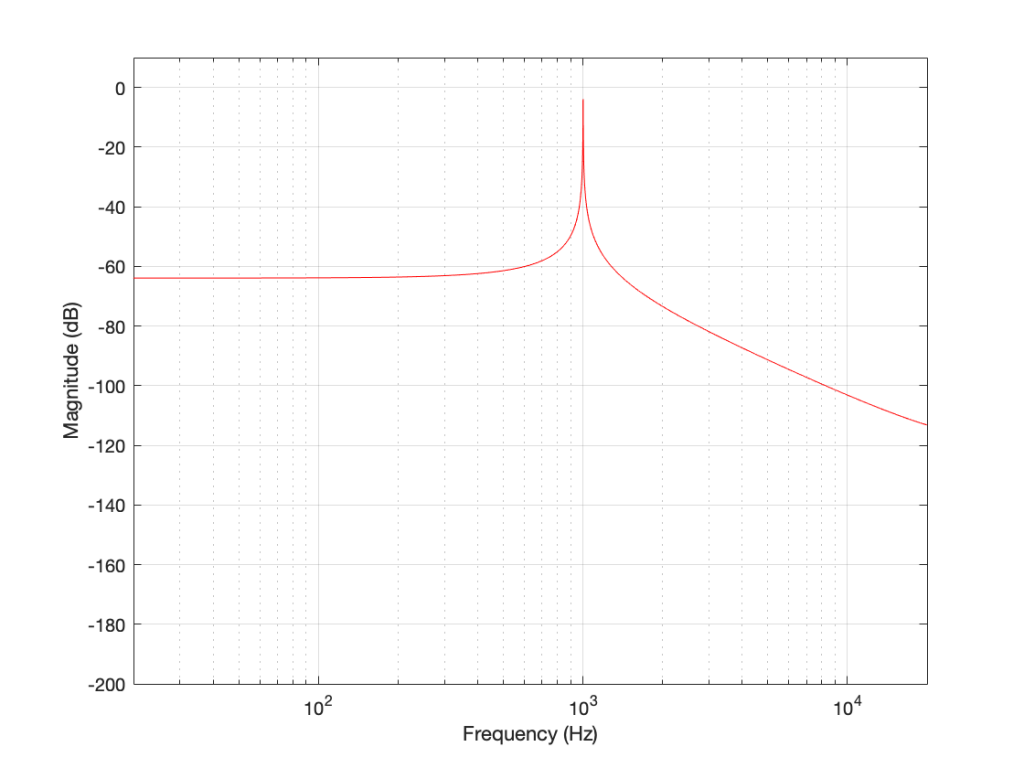Figure 2. The magnitude response of a 1000.5 Hz sine tone, sampled at 65,536 Hz, calculated using a 65,536-point DFT.

Now things don’t look so pretty. I can see that there’s signal around 1000 Hz, but it’s lower in level than the actual signal and there seems to be lots of stuff at other frequencies… Why is this?

In order to understand why the level in Figure 2 is lower than that in Figure 1, we have to zoom in at 1000 Hz and see the individual points on the plot.

As you can see in Figure 1 (Zoom), above, there is one DFT frequency “bin” at 1000 Hz, exactly where the sine wave is centred.

Figure 2 (Zoom) shows that, when the sine wave is at 1000.5 Hz, then the energy in that signal is distributed between two DFT frequency bins – at 1000 Hz and 1001 Hz. Since the energy is shared between two bins, then each of their level values is lower than the actual signal.

The reason for the “lots of stuff at other frequencies” problem is that the math in a DFT has a limited number of samples at its input, so it assumes that it is given a slice of time that repeats itself exactly.

## For example…

Let’s look at a portion of a plot like the one below:

If I asked you to continue this plot to the left and right (in other words, guess what’s under the gray rectangles), would you draw a curve like the one below?

This would be a good guess. However, the figure below is also a good guess.

Of course, we could guess something else. Perhaps Figure 3 is mostly correct, but we should add a drawing of Calvin and Hobbes on a toboggan, sliding down the hill to certain death as well. You never know what was originally behind those grey rectangles…

This is exactly the problem the math behind a DFT has – you feed it a “slice” of a recording, some number of samples long, and the math (let’s call it “a computer”, since it’s probably doing the math) has to assume that this slice is a portion of time that is repeated forever – it started at the beginning of time, and it will continue repeating until the end of time. In essence, it has to make an “extrapolation” like the one shown in Figure 4 because it doesn’t have enough information to make assumptions that result in the plot in Figure 3.

## For example: Part 2

Let’s go back to the bell recording that we’ve been looking at in the previous posts. We have a portion of a recording, 2048 samples long. If I plot that signal, it looks like the curve in Figure 5.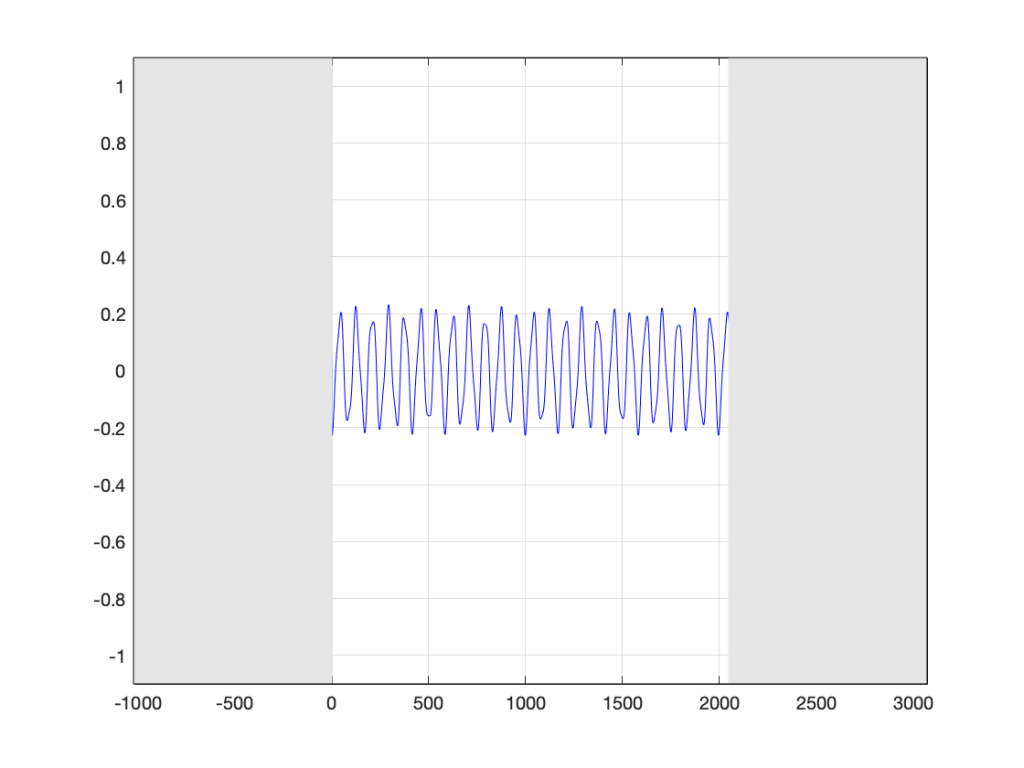Figure 5. The bell recording we saw in previous postings, hiding the information that comes before and after.

When the computer does the DFT math, the assumption is that this is a slice that is repeated forever. So, the computer’s assumption is that the original signal looks like the one below, in Figure 6.

I’ve put rectangles around the beginning (at sample 1) and end (at sample 2048) of the slice to highlight what the signal looks like, according to the computer… The signal in the left half of the left rectangle (ending at sample 0) is the end of the slice of the recording, right before it repeats. The signal starting at 2049 is the beginning again – a repeat of sample 1.

If we zoom in on the signal in the left rectangle, it looks like Figure 7.

Notice that vertical line at sample 1 (actually going from sample 0 to sample 1, to be accurate). Of course, our original bell recording didn’t have that “instantaneous” drop in there – but the computer assumes it does because it doesn’t have enough information to assume anything else.

If we wanted to actually make that “instantaneous” vertical change in the signal (with a theoretical slope of infinity – although it’s not really that steep….), we would have to add other frequencies to our original signal. Generally, you can assume that, the higher the slope of an audio signal, either 1) the louder the signal or 2) the more high frequency content in the signal. Let’s look at the second one of those.

Let’s look at portions of sine waves at three different frequencies. These are shown below, in Figure 8. The top plot shows a sine wave with some frequency, showing how it looks as it passes phase = 0º (which we’ll call “time = 0” (on the X-axis)). At that moment, the sine wave has a value of 0 (on the Y-axis) and the slope is positive (it’s going upwards). The middle plot shows a sine wave with 3 times the frequency (notice that there are 6 negative-and-positive bumps in there instead of just 2). Everything I said about the top plot is still true. The level is 0 at time=0, and the slope is positive. The bottom plot is 5 times the frequency (10 bumps instead of 2). And, again, at time=0, everything is the same.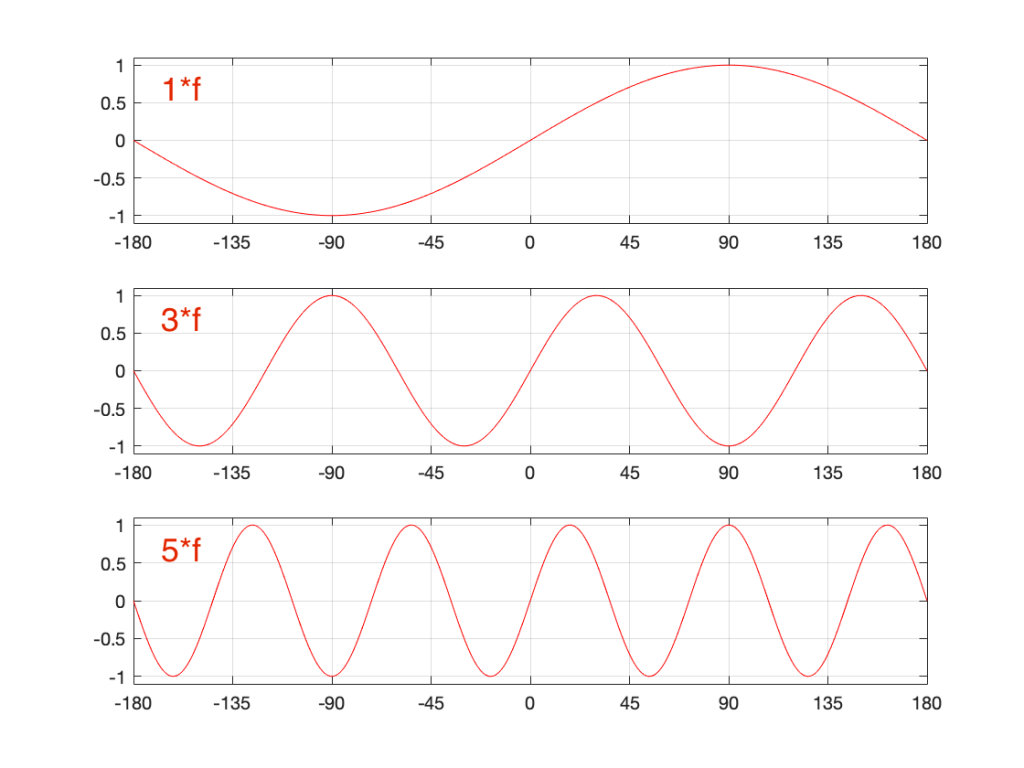Figure 8. Three sinusoidal waves at related frequencies. We’re looking at the curves as they cross time=0 (on the X-axis).

Let’s look a little more carefully at the slope of the signal as it crosses time=0. I’ve added blue lines in Figure 9 to highlight those.

Notice that, as the frequency increases, the slope of the signal when it crosses the 0 line also increases (assuming that the maximum amplitude stays the same – all three sine waves go from -1 to 1 on the Y-axis.

One take-away from that is the idea that I’ve already mentioned: the only way to get a steep slope in an audio signal is to add high frequency content. Or, to say it another way: if your audio signal has a steep slope at some time, it must contain energy at high frequencies.

Although I won’t explain here, the truth is just a little more complicated. This is because what we’re really looking for is a sharp change in the slope of the signal – the “corners” in the plot around Sample 0 in Figure 7. I’ve put little red circles around those corners to highlight them, shown below in Figure 10. When audio geeks see a sharp corner like that in an audio signal, they say that the waveform is discontinuous – meaning that the level jumps suddenly to something unexpected – which means that its slope does as well.

Basically, if you see a discontinuity in an audio signal that is otherwise smooth, you’re probably going to hear a “click”. The audibility of the click depends on how big a jump there is in the signal relative to the remaining signal. (For example, if you put a discontinuity in a nice, smooth, sine wave, you’ll hear it. If you put a discontinuity in a white noise signal – which is made up of nothing but discontinuities (because it’s random) then you won’t hear it…)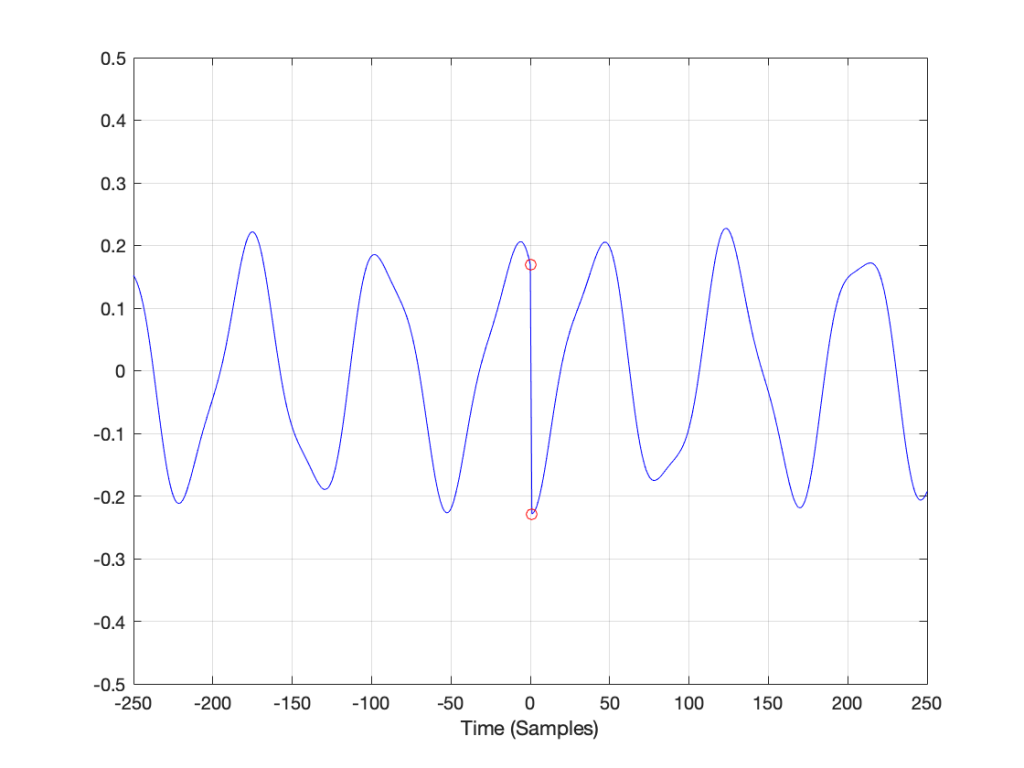Figure 10. The red circles show the discontinuities in the slope of the signal when it is assumed that it repeats.

## Circling back…

Think back to the examples I started with at the beginning of this post. When I do a 65,536-point DFT of a 1000 Hz sine wave sampled at 65,536 Hz, the result is a nice clean-looking magnitude response (Figure 1). However, when I do a 65,536-point DFT of a 1000.5 Hz sine wave sampled at 65,536 Hz, the result is not nearly as nice. Why?

Think about how the end of the two sine waves join up with their beginnings. When you do a 65,536-point DFT on a signal that has a sampling rate of 65,536 Hz, then the slice of time that you’re analysing is exactly 1 second long. A 1000 Hz sine wave, repeats itself exactly after 1 second, so the 65,537th sample is identical to the first. If you join the last 30 samples of the slice to the first 30 samples, it will look like the red curve on the top plot in Figure 10, below.

However, if the sinusoid has a frequency of 1000.5 Hz, then it is only half-way through the waveform when you get to the end of the second. This will look like the lower black curve in Figure 10.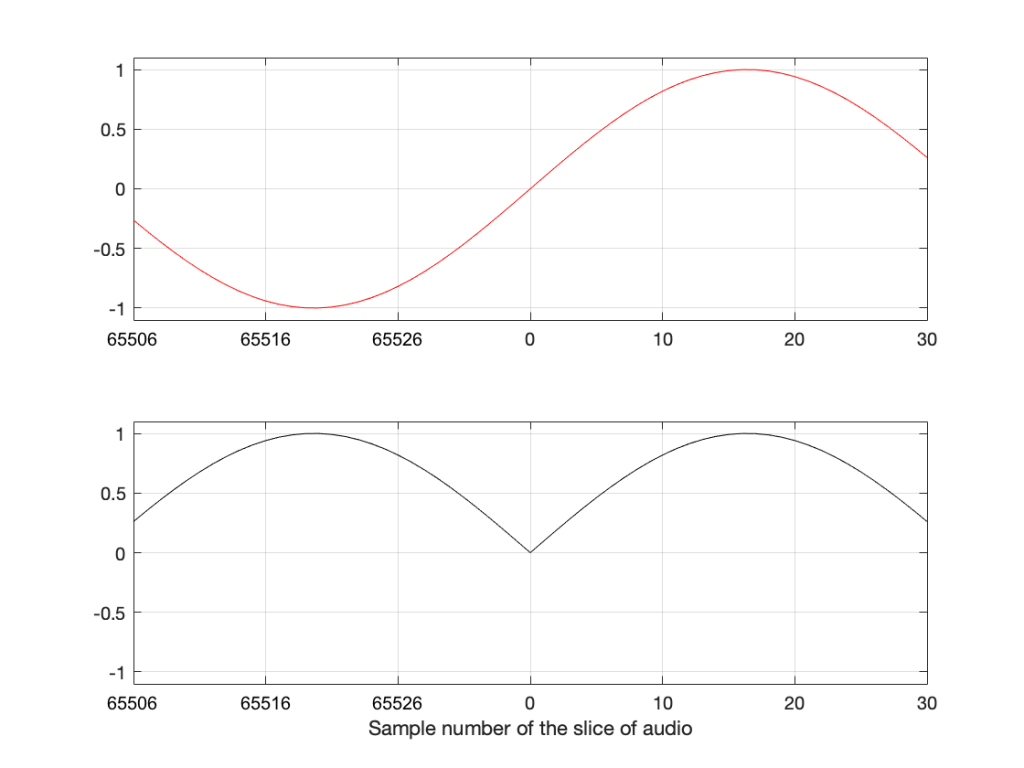Figure 11. The top plot shows the a 1000 Hz sine wave at the end of exactly 1 second, joined to the beginning of the same sine wave. The bottom plot shows the same for a 1000.5 Hz sine wave

Notice that the lower plot has a discontinuity in the slope of the waveform. This means that there is energy in frequencies other than 1000.5 Hz in it. And, in fact, if you measured how much energy there is in that weird waveform that sounds like a sine wave most of the time, but has a little click every second, you’ll find out that the result is already plotted in Figure 2.

## The conclusion

The important thing to remember from this posting is that a DFT tells you what the relative frequency content of the signal is – but only for the signal that you give it. And, in most cases, the signal that you give it (a slice of time that is looped for infinity) is not the same as the total signal that you took the slice from.

So, most of the time, a DFT (or FFT – you choose what you call it) is NOT showing you what is in your signal in real life. It’s just giving you a reasonably good idea of what’s in there – and you have to understand how to interpret the plot that you’re looking at.

In other words, Figure 2 does not show me how a 1000.5 Hz sine tone sounds – but Figure 1 shows me how a 1000 Hz sine tone sounds. However, Figures 1 and 2 show me exactly how the computer “hears” those signals – or at least the portion of audio that I gave it to listen to.

There is a general term applied to the problem that we’re talking about. It’s called “windowing effects” because the DFT is looking at a “window” of time (up to now, I’ve been calling it a “slice” of the audio signal. I’m going to change to using the word “time window” or just “window” from now on.

In the next posting, DFT’s Part 5: Windowing, we’ll look at some sneaky ways to minimise these windowing effects so that they’re less distracting when you’re looking at magnitude response plots.